The purpose of this vignette is to display all high-level plots available in GGally to be used in particular with ggduo() and ggpairs(). The name of all the corresponding functions are of the form ggally_*(). Most of them accept a discrete variables to be passed to the colour aesthetic.

We can distinct bivariate plots requiring two variables for x and y axis respectively and diagonal plots when the same variable is plotted on x and y axis.

library(GGally, quietly = TRUE)
#> Registered S3 method overwritten by 'GGally':
#>   method from
#>   +.gg   ggplot2
data(tips)

## Bivariate plots

### with 2x continuous variables

#### ggally_autopoint()

ggally_autopoint(tips, aes(x = total_bill, y = tip))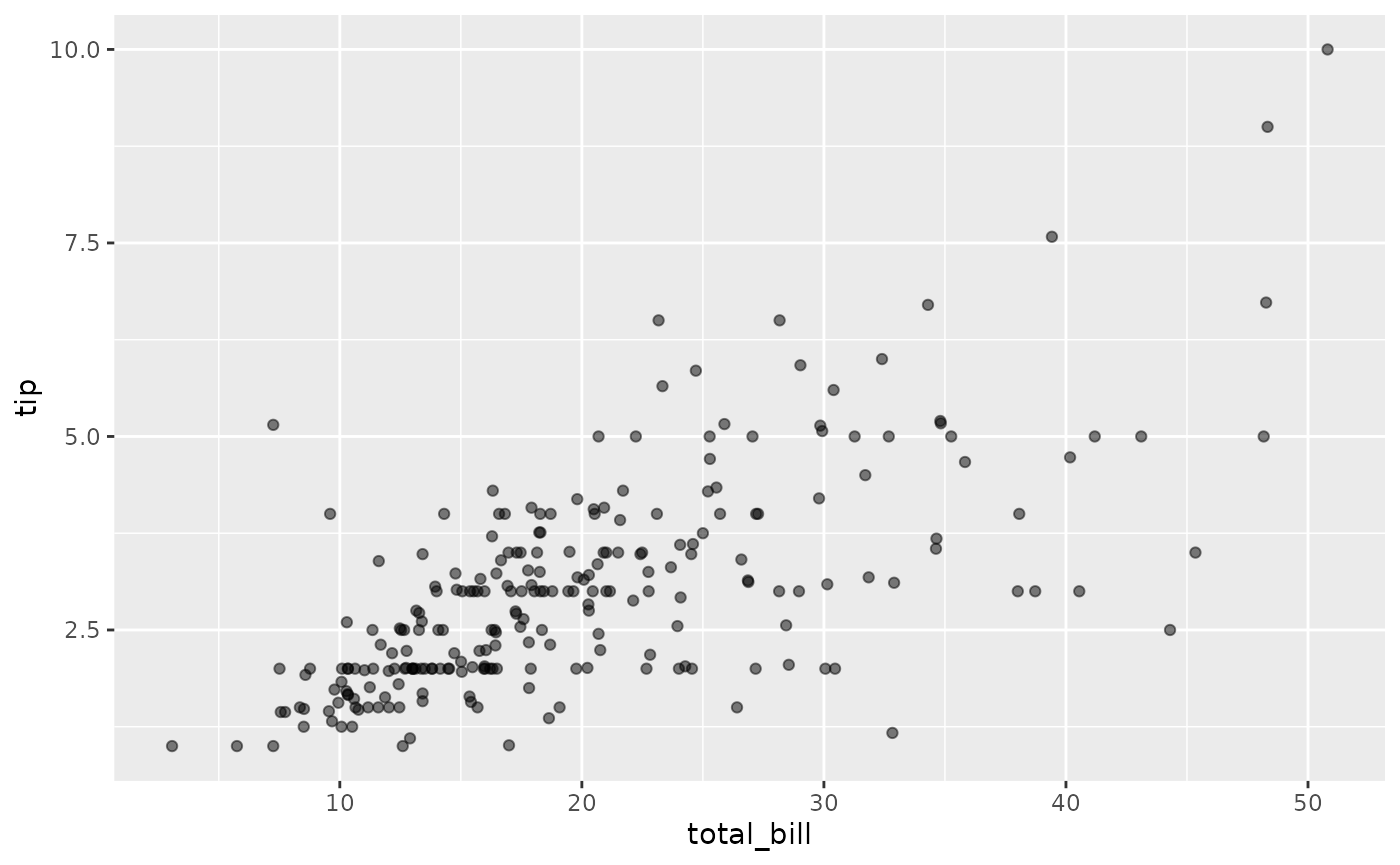ggally_autopoint(tips, aes(x = total_bill, y = tip, colour = time))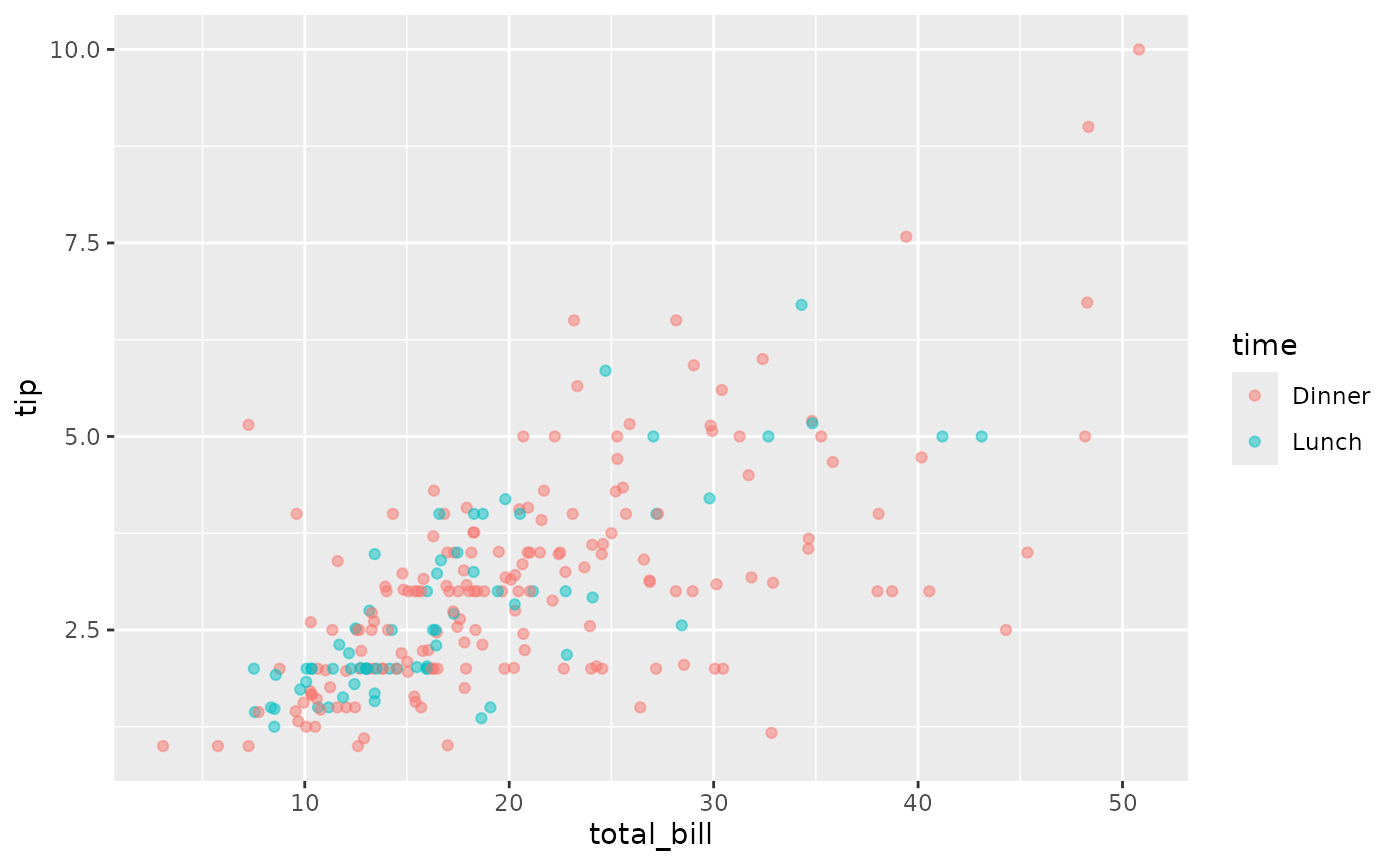#### ggally_cor()

ggally_cor(tips, aes(x = total_bill, y = tip))ggally_cor(tips, aes(x = total_bill, y = tip, colour = time))See also ggally_statistic().

#### ggally_density()

ggally_density(tips, aes(x = total_bill, y = tip))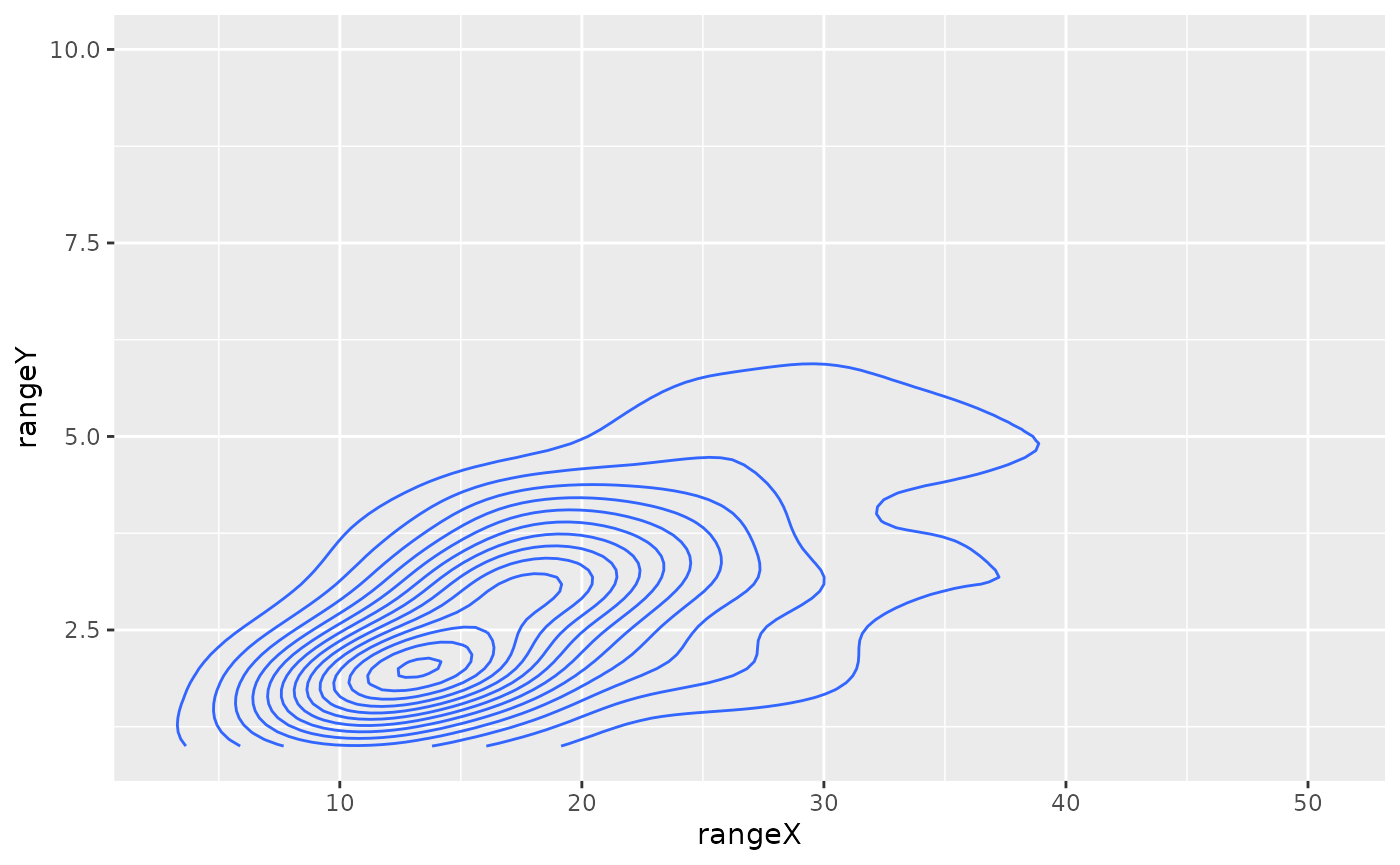ggally_density(tips, aes(x = total_bill, y = tip, colour = time))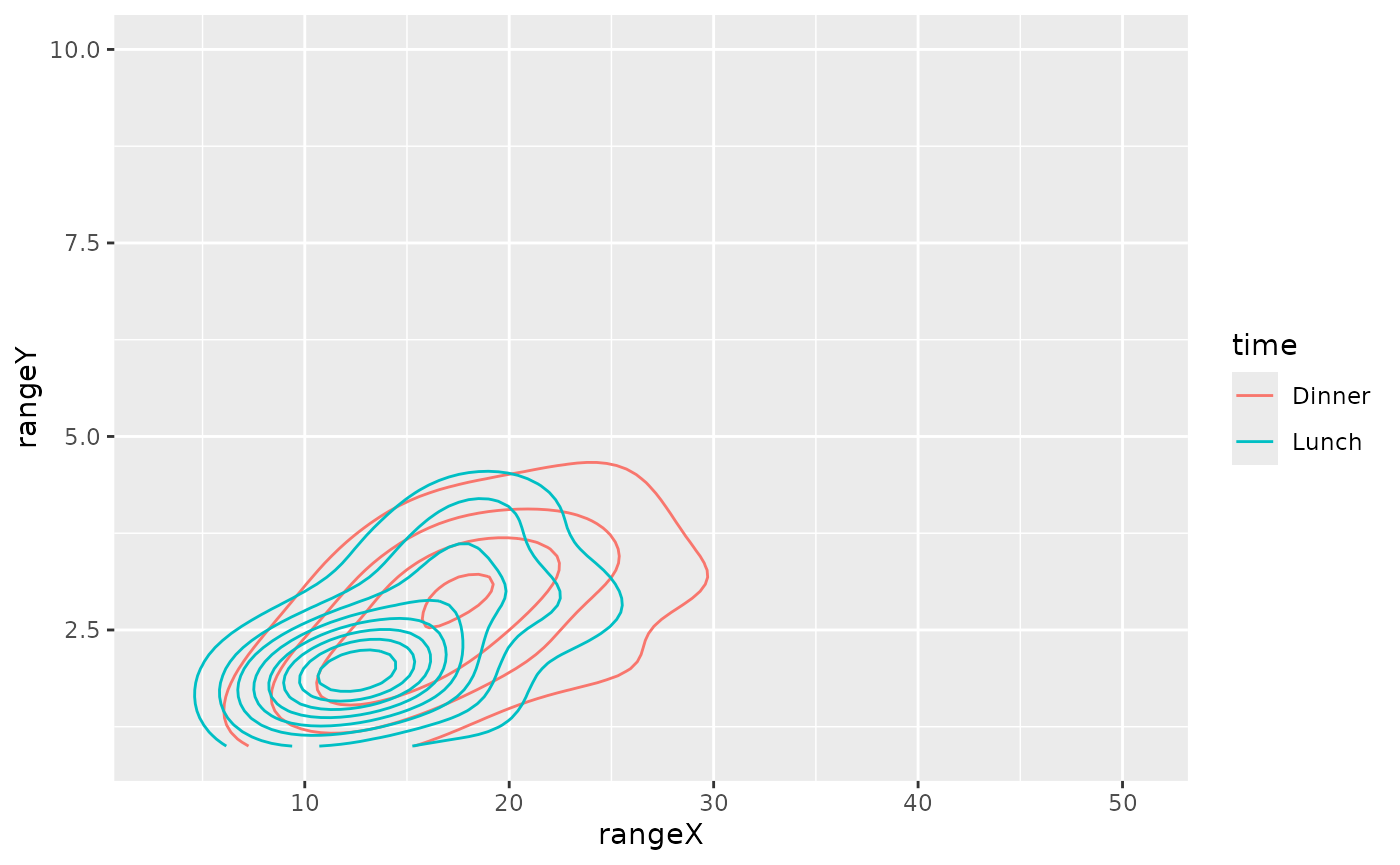#### ggally_points()

ggally_points(tips, aes(x = total_bill, y = tip))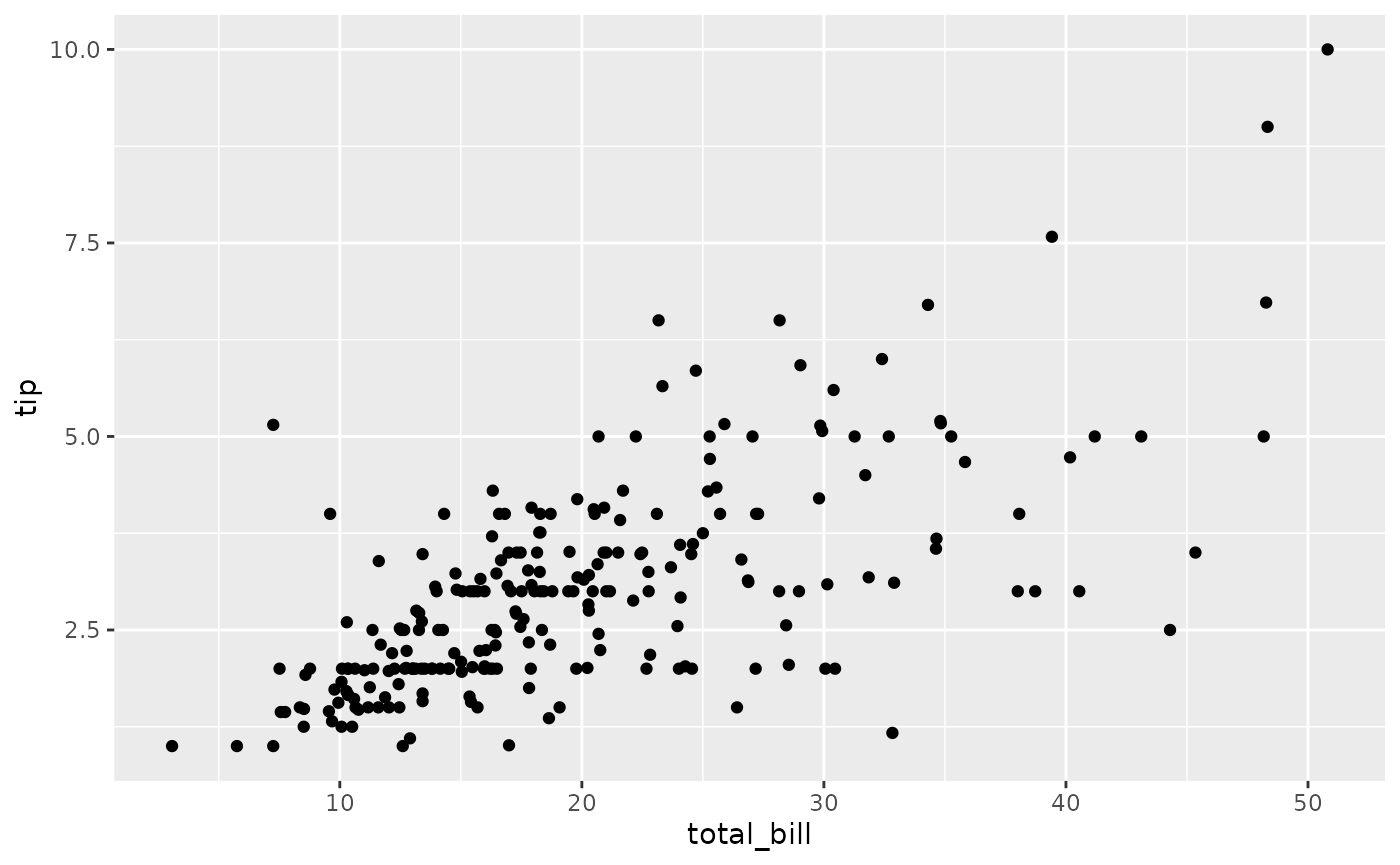ggally_points(tips, aes(x = total_bill, y = tip, colour = time))#### ggally_smooth(), ggally_smooth_lm() & ggally_smooth_loess()

ggally_smooth_lm(tips, aes(x = total_bill, y = tip))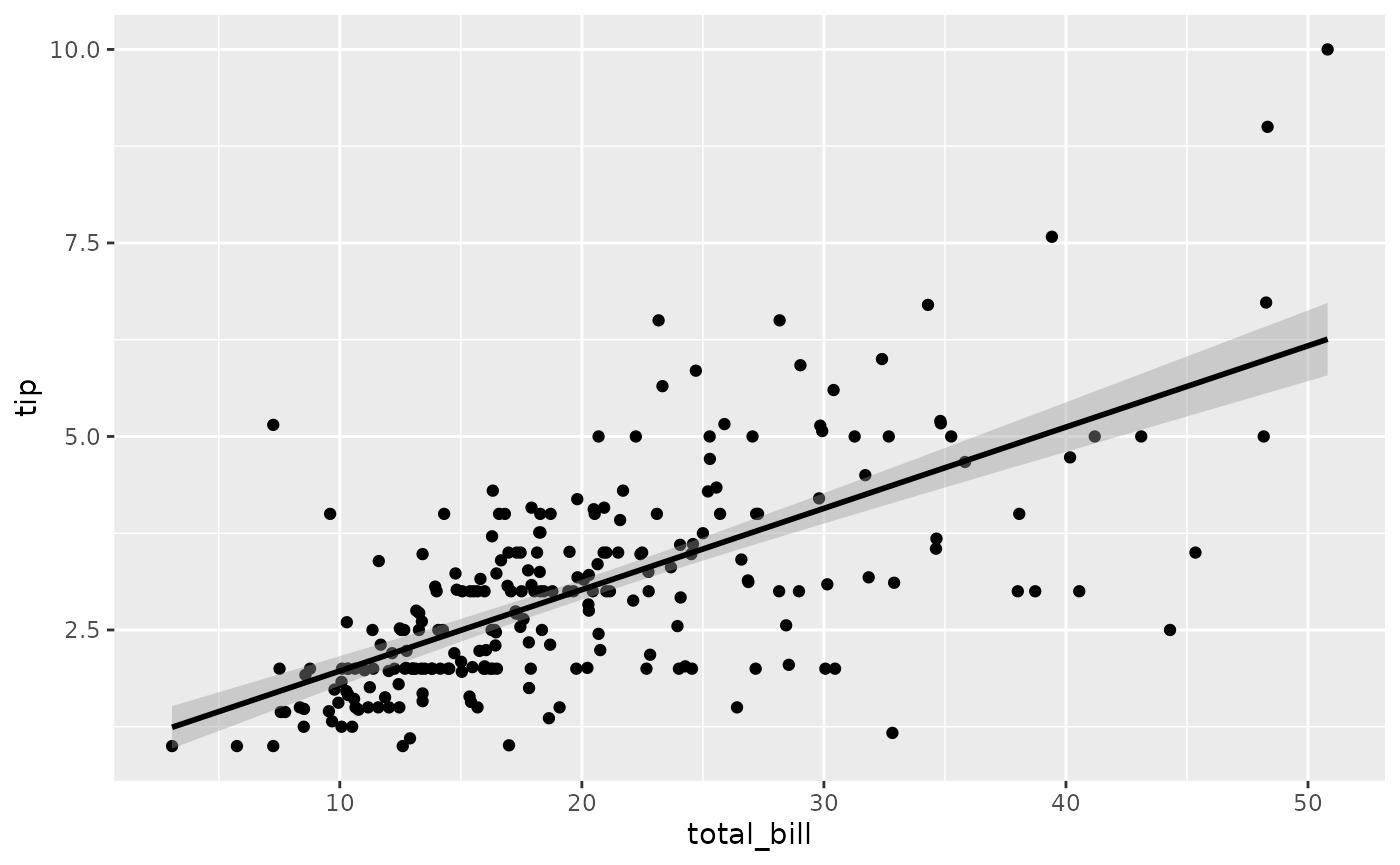ggally_smooth_lm(tips, aes(x = total_bill, y = tip, colour = time))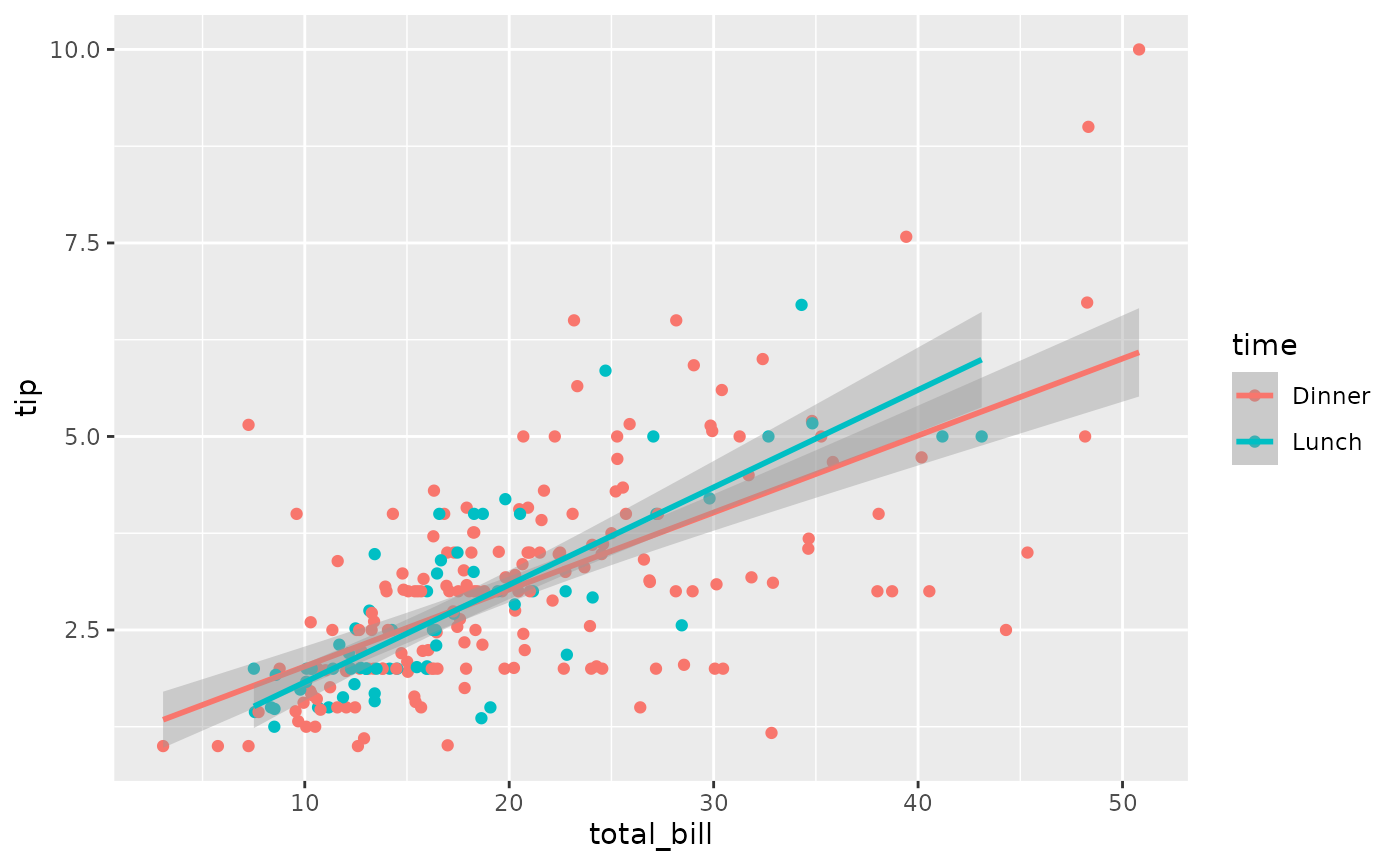ggally_smooth_loess(tips, aes(x = total_bill, y = tip))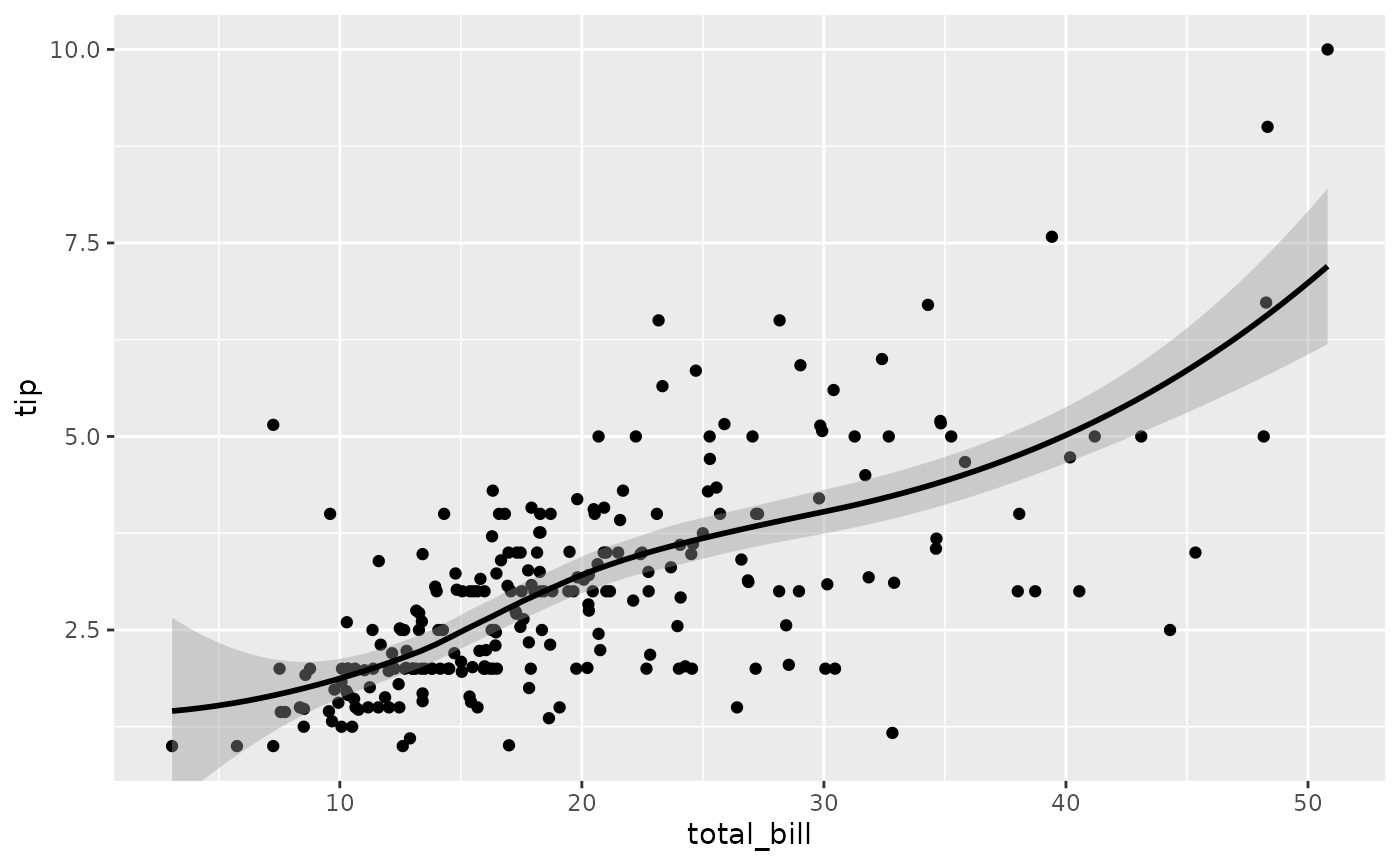ggally_smooth_loess(tips, aes(x = total_bill, y = tip, colour = time))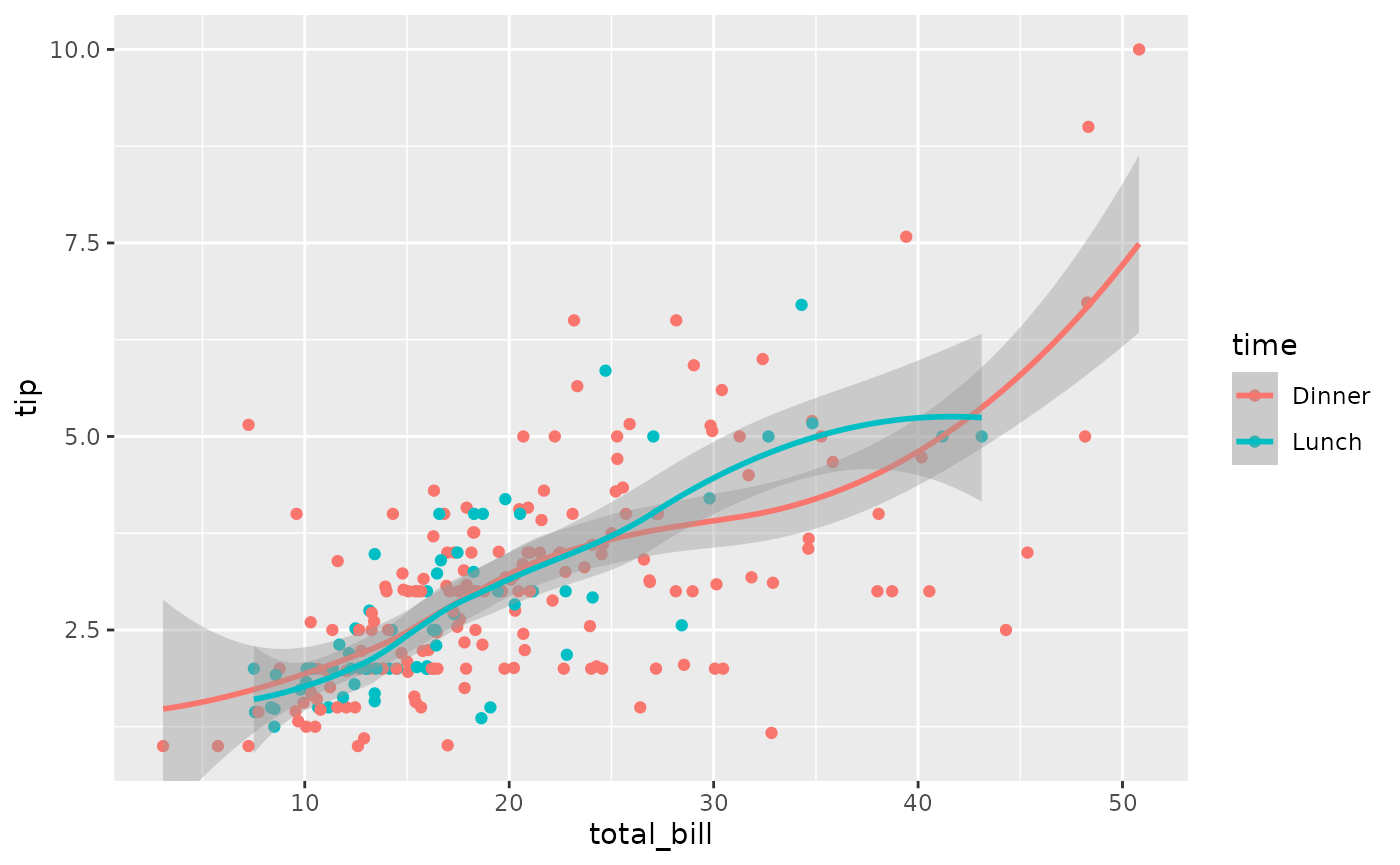See also ggally_smooth() for more options.

### with 2x discrete variables

#### ggally_colbar()

ggally_colbar(tips, aes(x = day, y = smoker))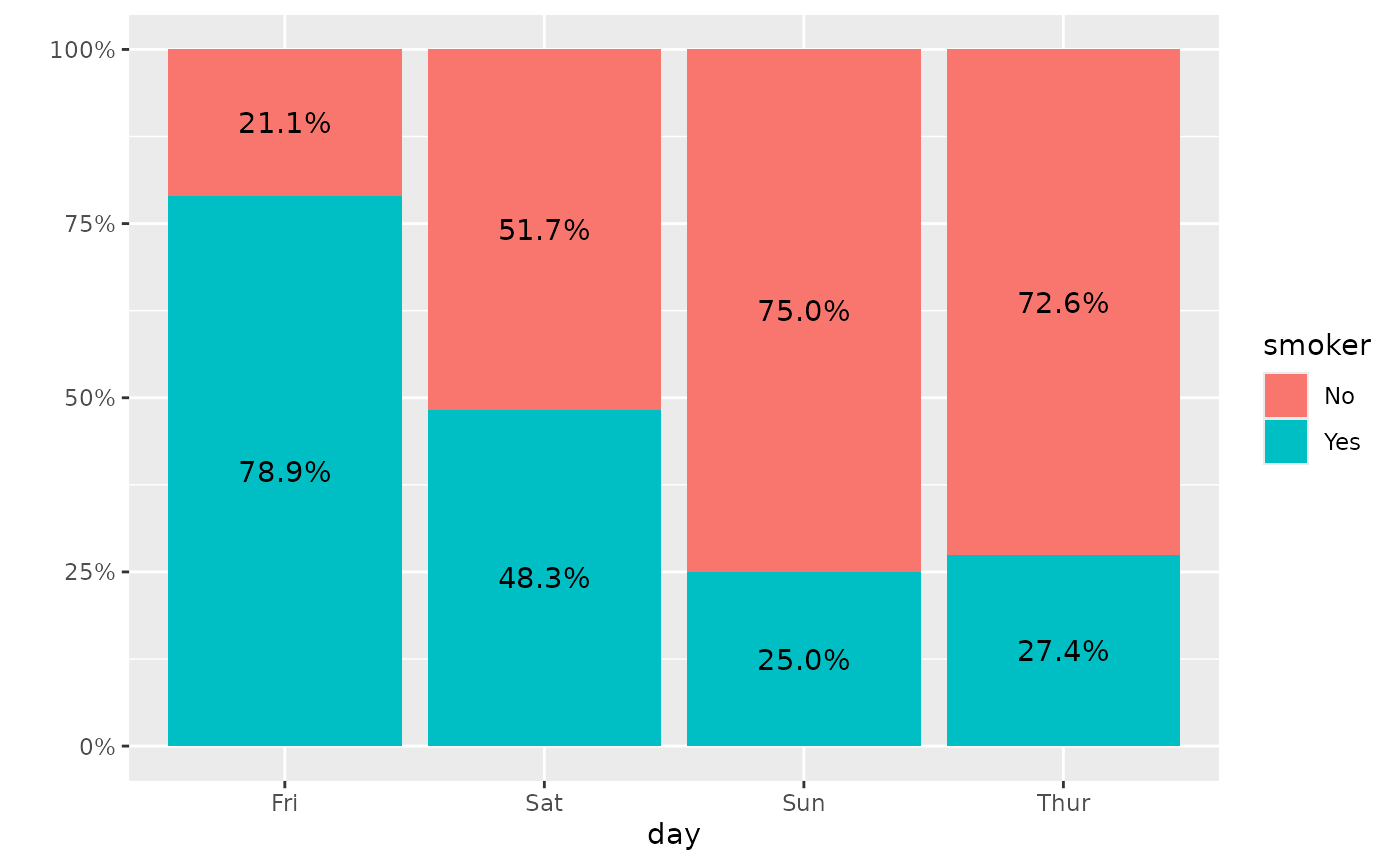Note: the colour aesthetic is not taken into account.

#### ggally_autopoint()

ggally_autopoint(tips, aes(x = day, y = smoker))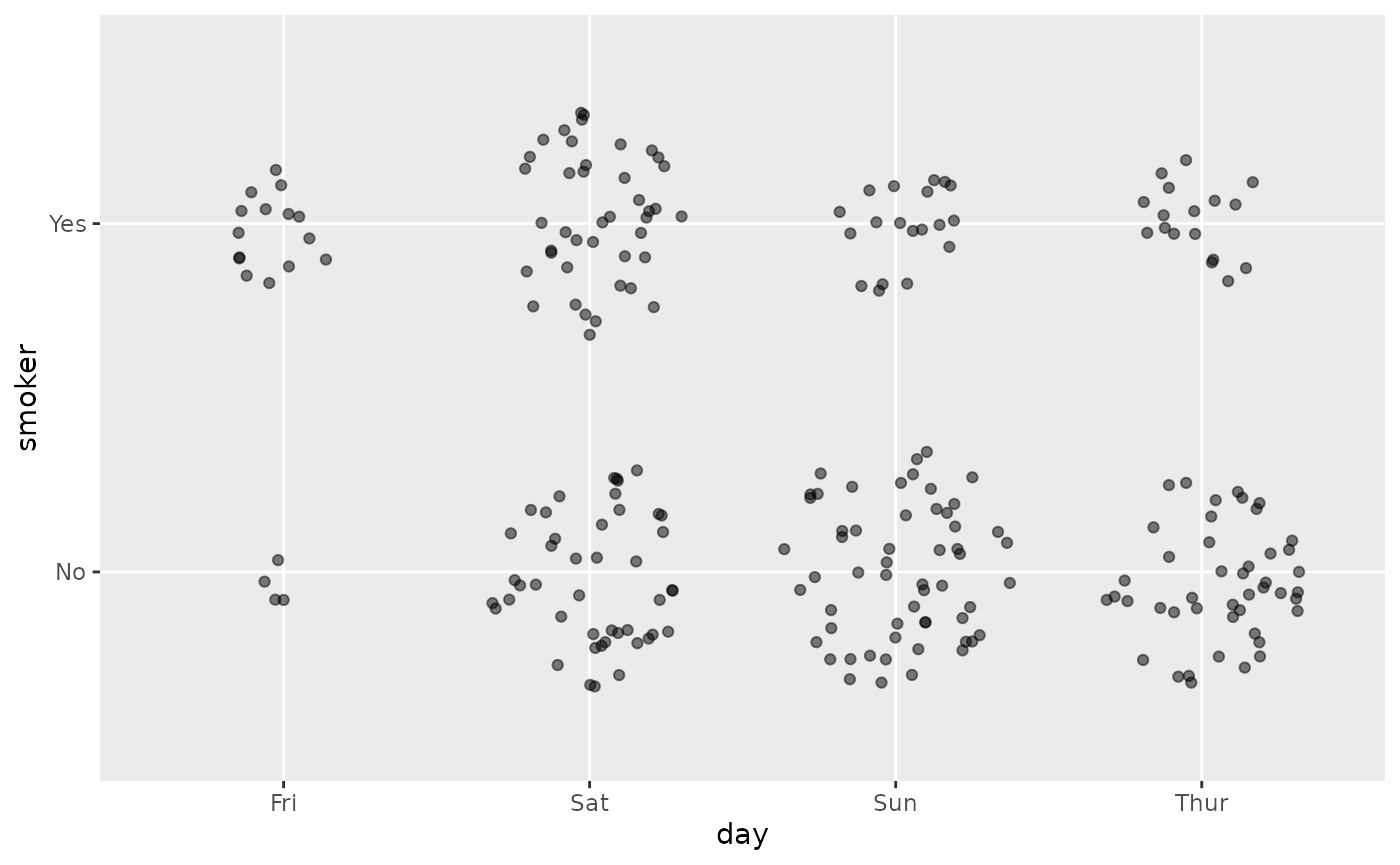ggally_autopoint(tips, aes(x = day, y = smoker, colour = time))#### ggally_count()

ggally_count(tips, aes(x = day, y = smoker))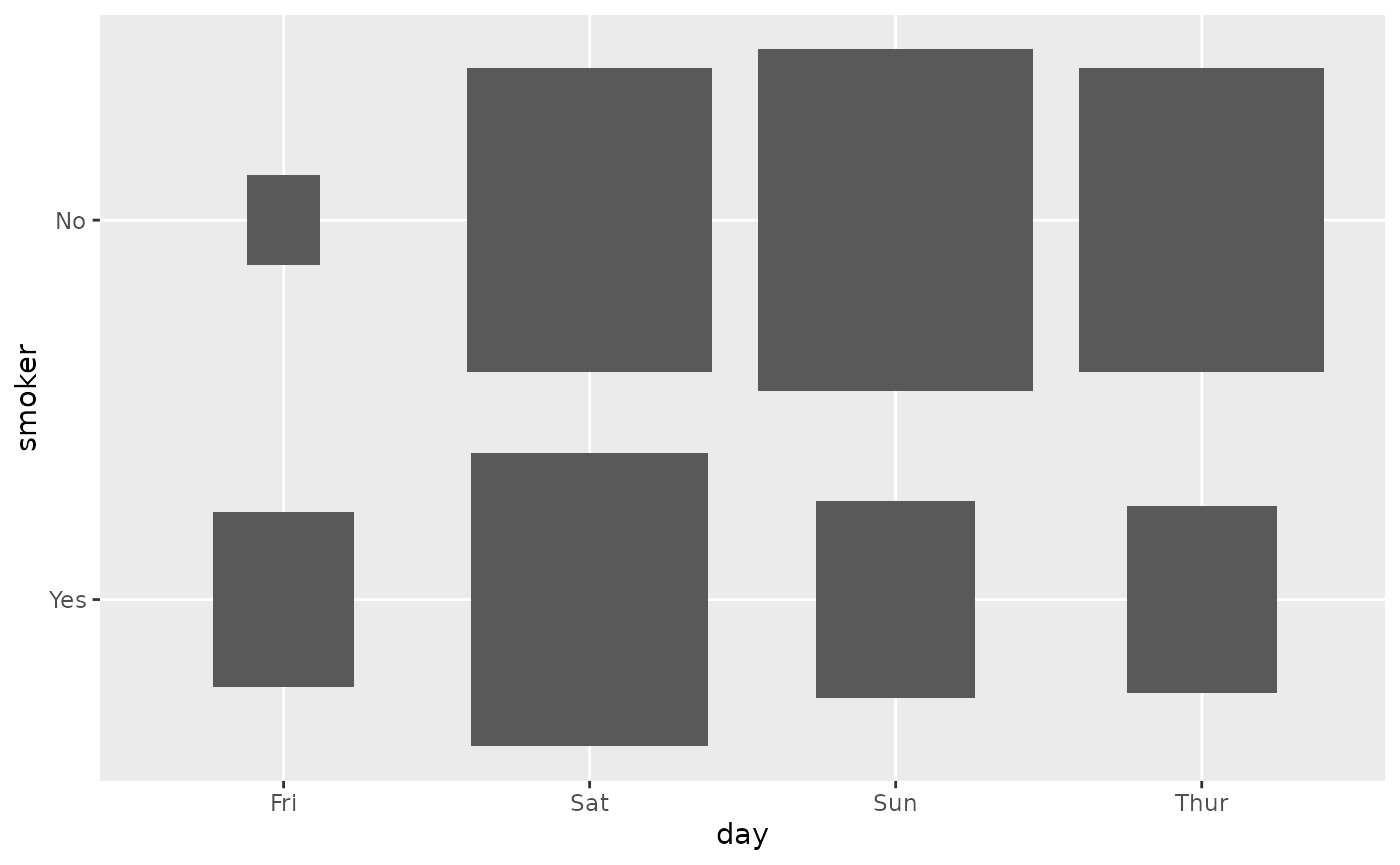ggally_count(tips, aes(x = day, y = smoker, colour = time))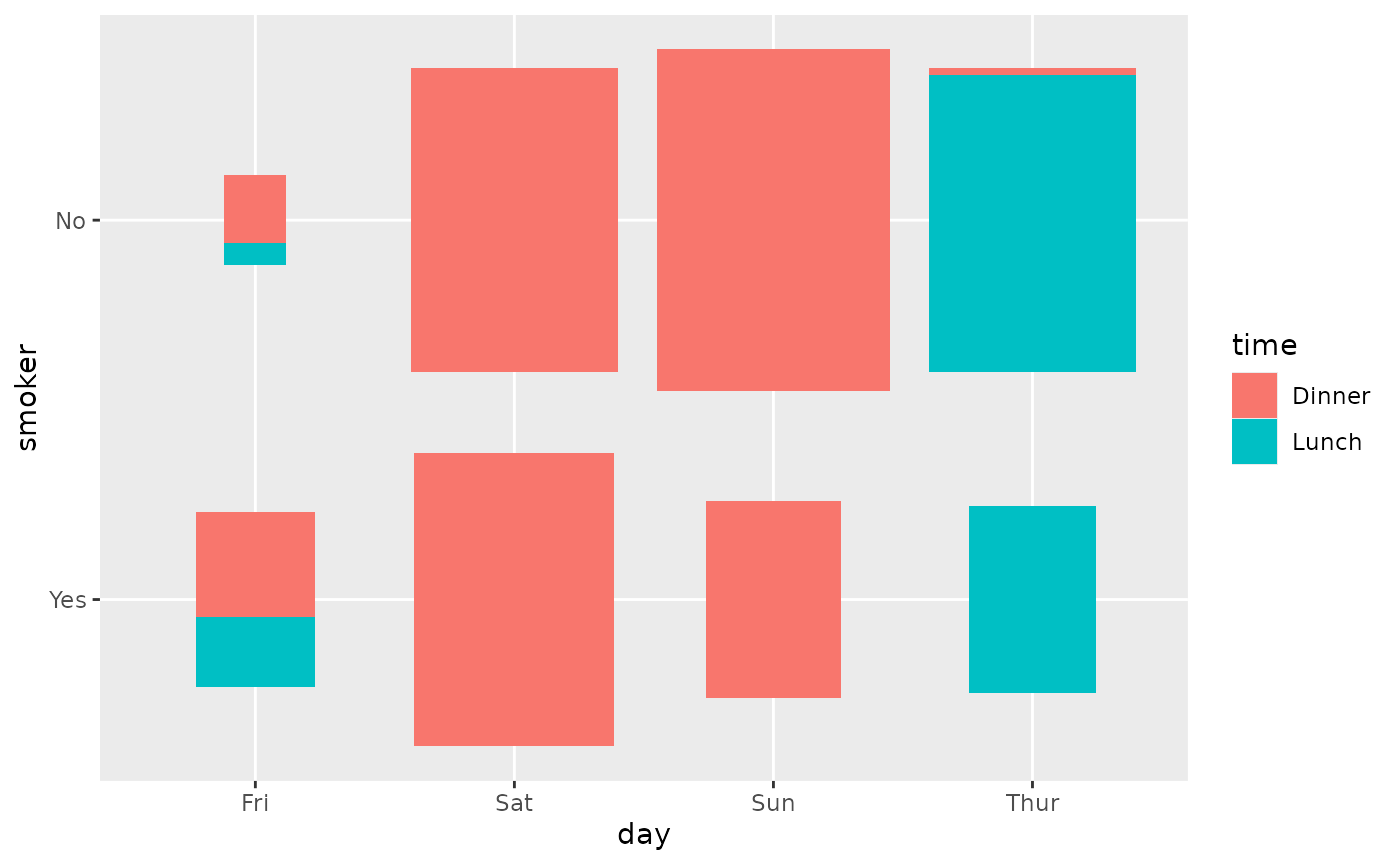#### ggally_cross()

ggally_cross(tips, aes(x = day, y = smoker))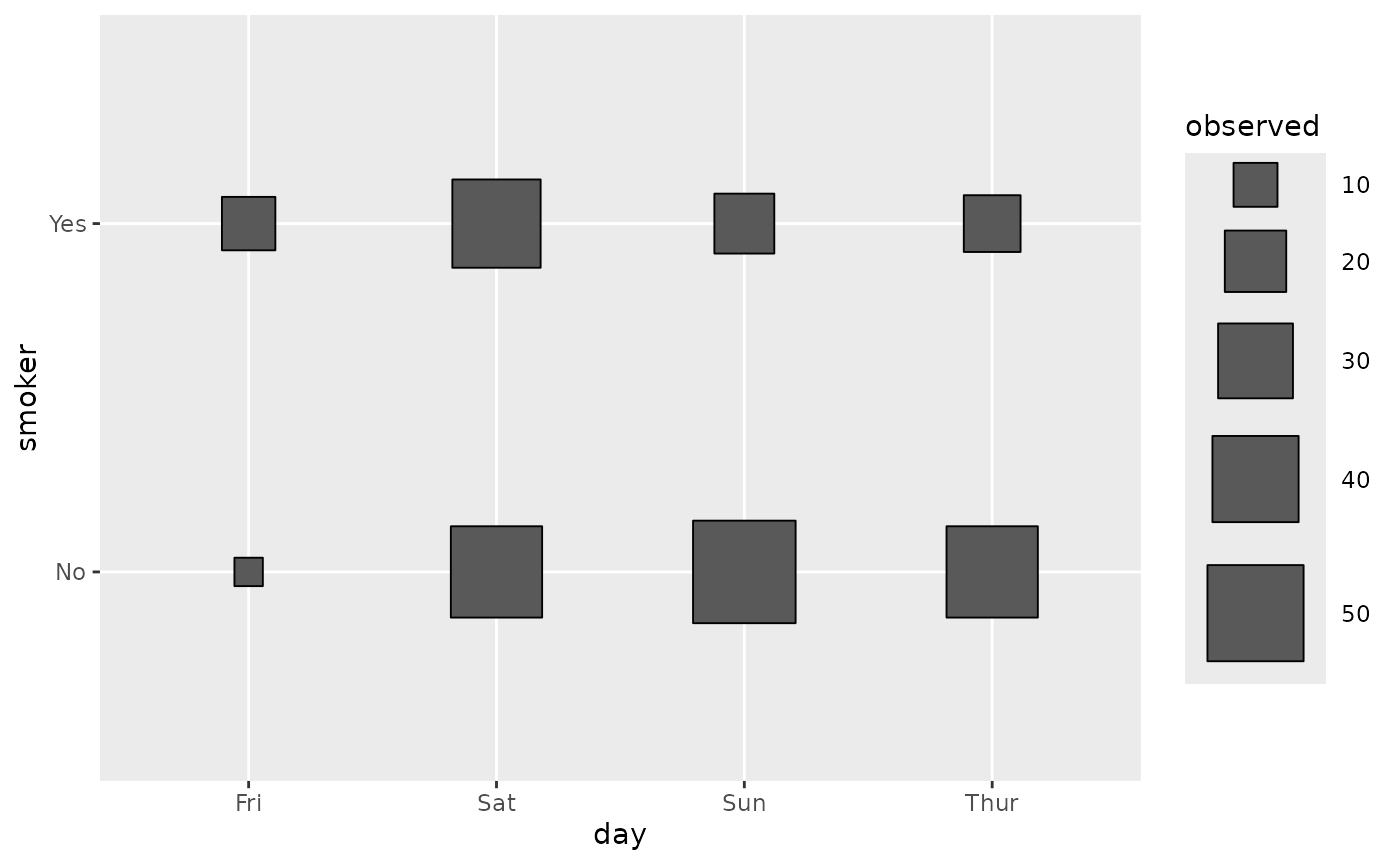ggally_cross(tips, aes(x = day, y = smoker, colour = time))ggally_cross(tips, aes(x = day, y = smoker, colour = smoker))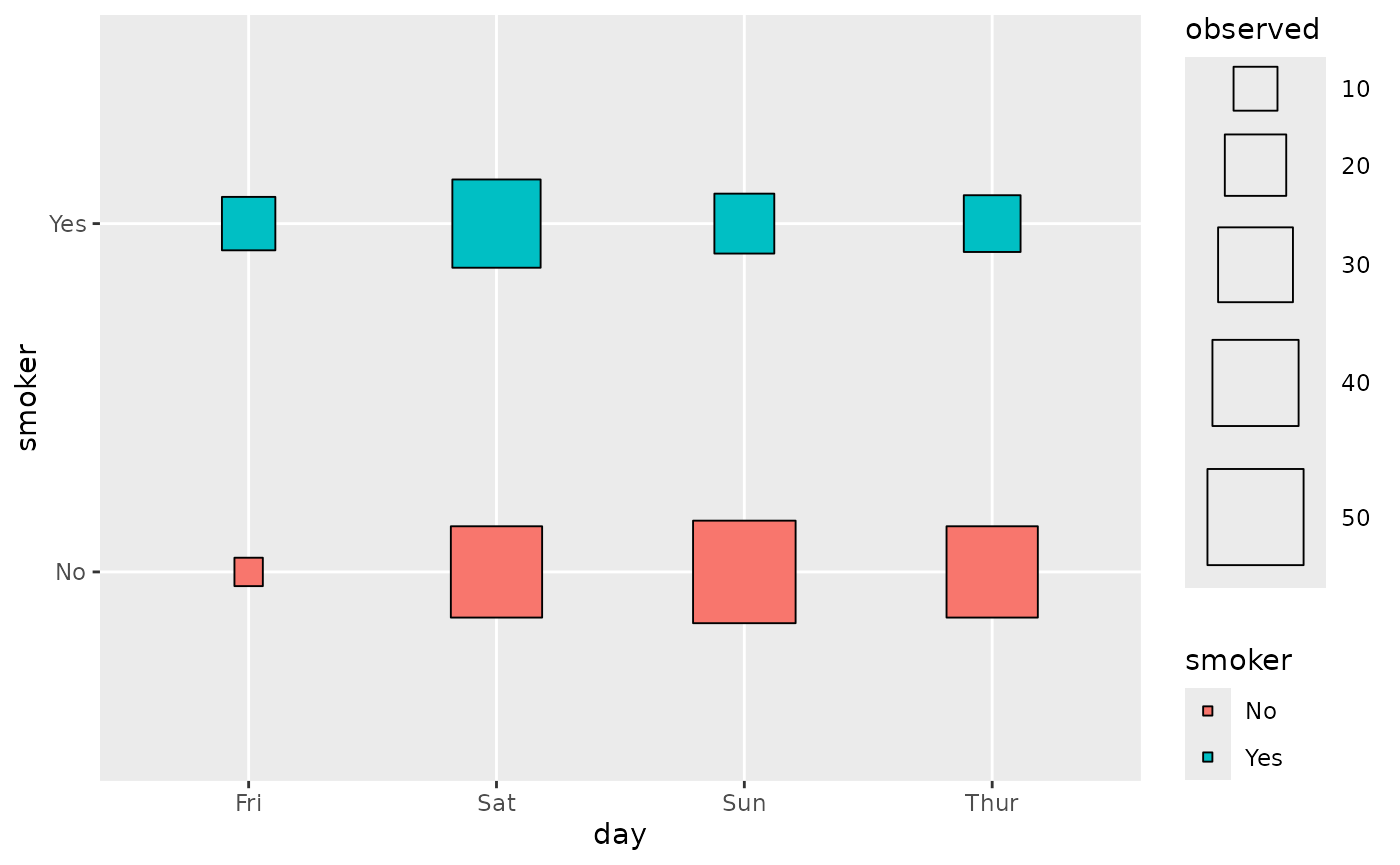Note: colour aesthetic is taken into account only if it corresponds to x or to y.

#### ggally_crosstable()

ggally_crosstable(tips, aes(x = day, y = smoker))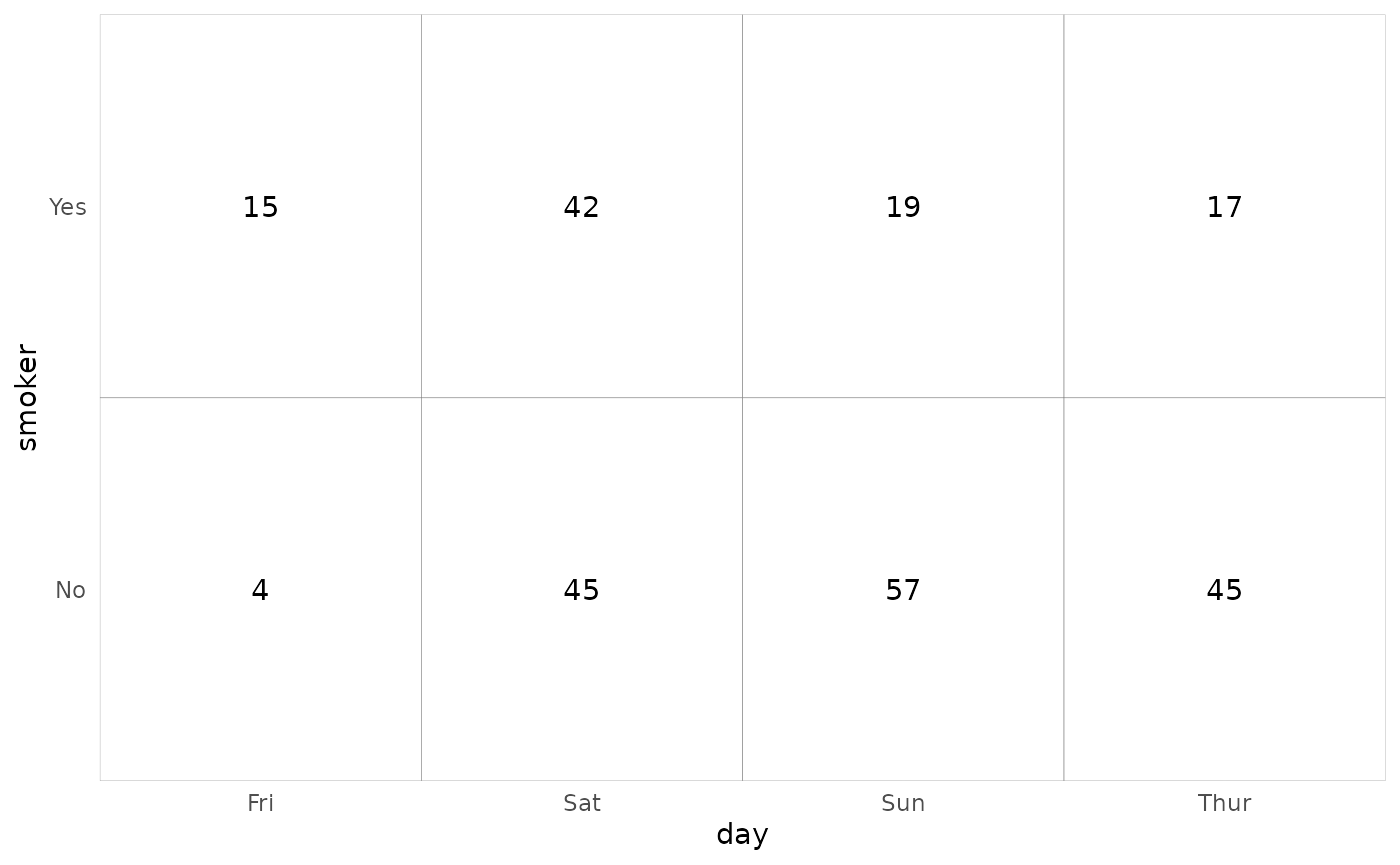ggally_crosstable(tips, aes(x = day, y = smoker), cells = "col.prop", fill = "std.resid")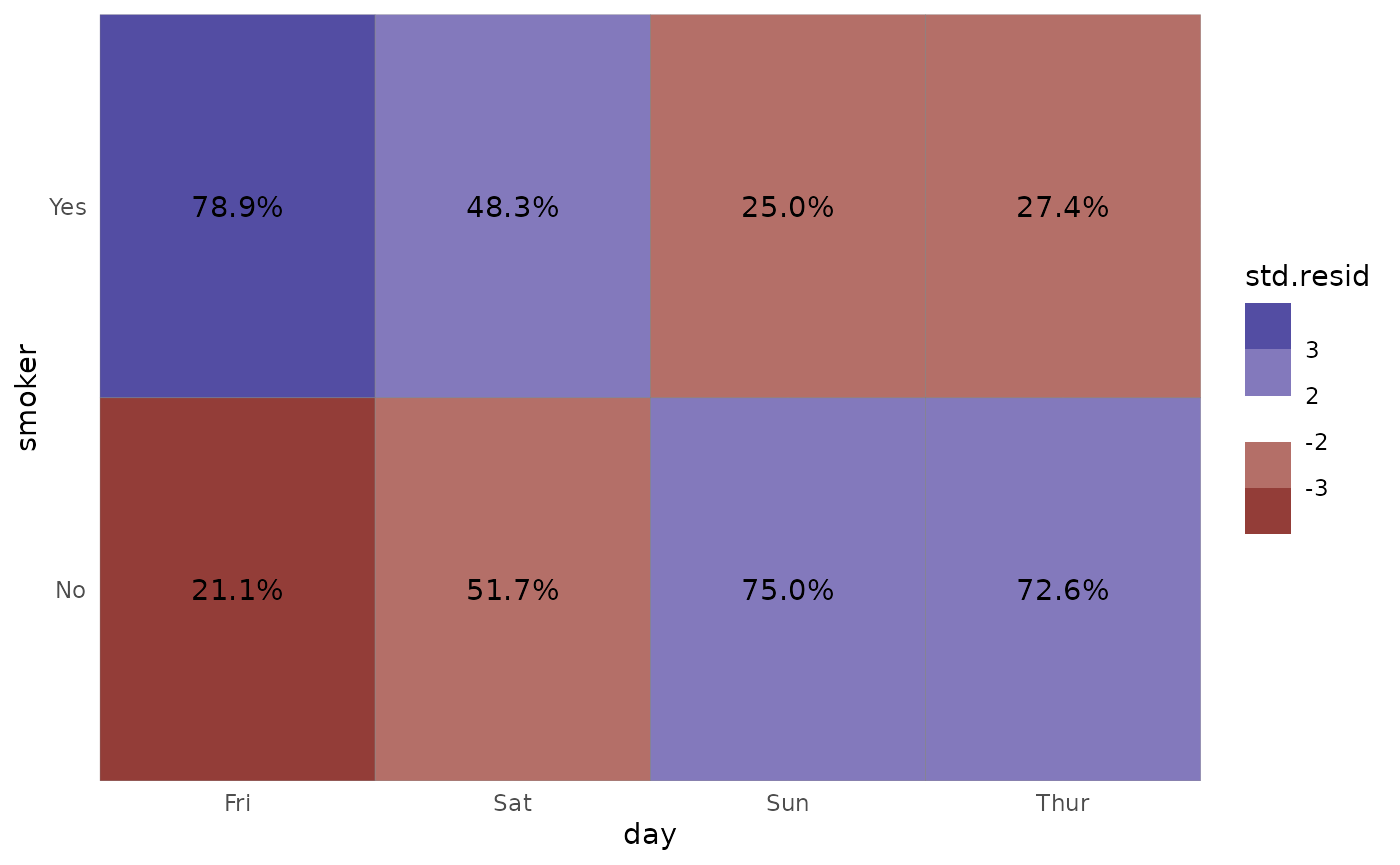Note: colour aesthetic is not taken into account.

#### ggally_facetbar()

ggally_facetbar(tips, aes(x = day, y = smoker))ggally_facetbar(tips, aes(x = day, y = smoker, colour = time))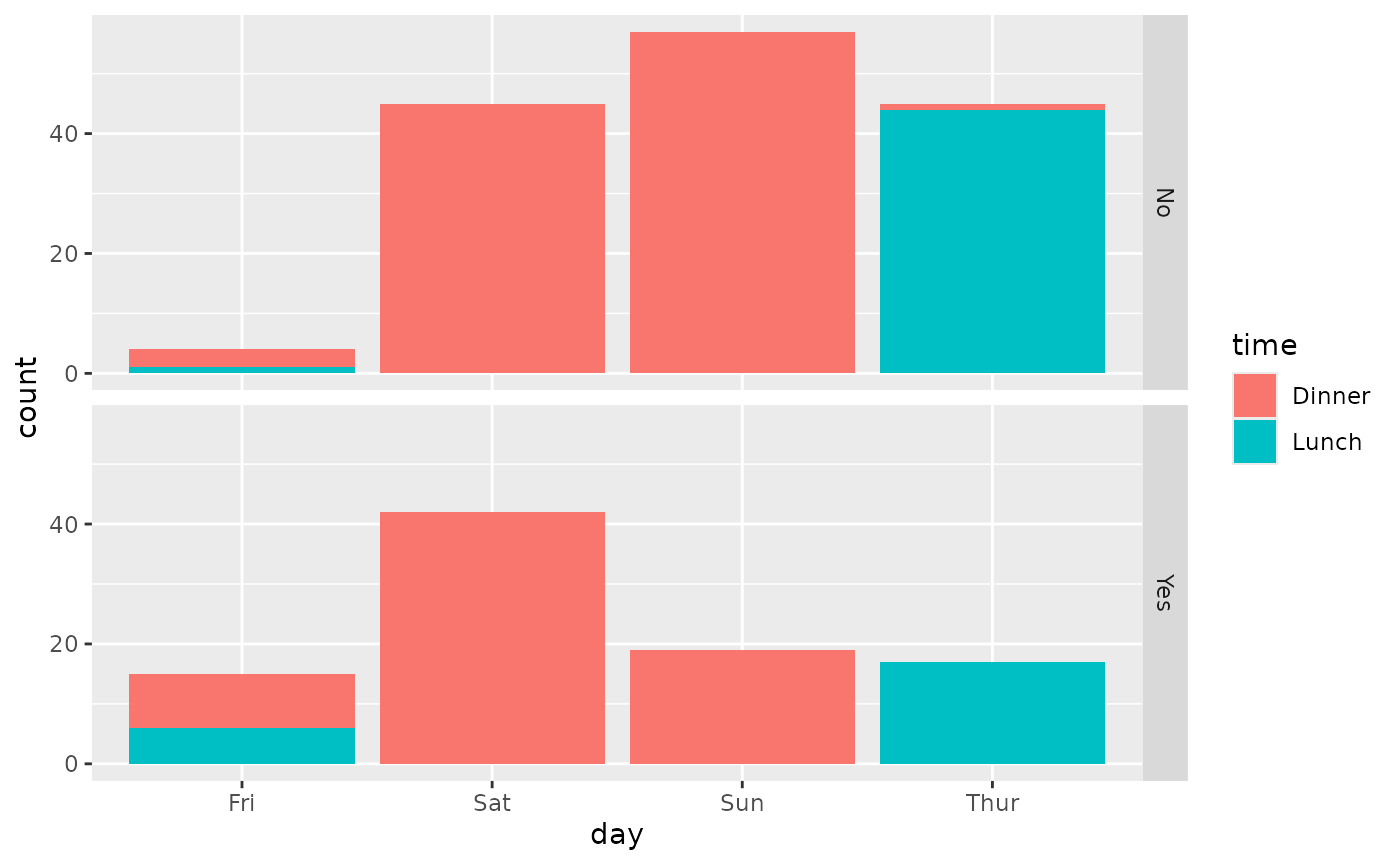#### ggally_ratio()

ggally_ratio(tips, aes(x = day, y = smoker))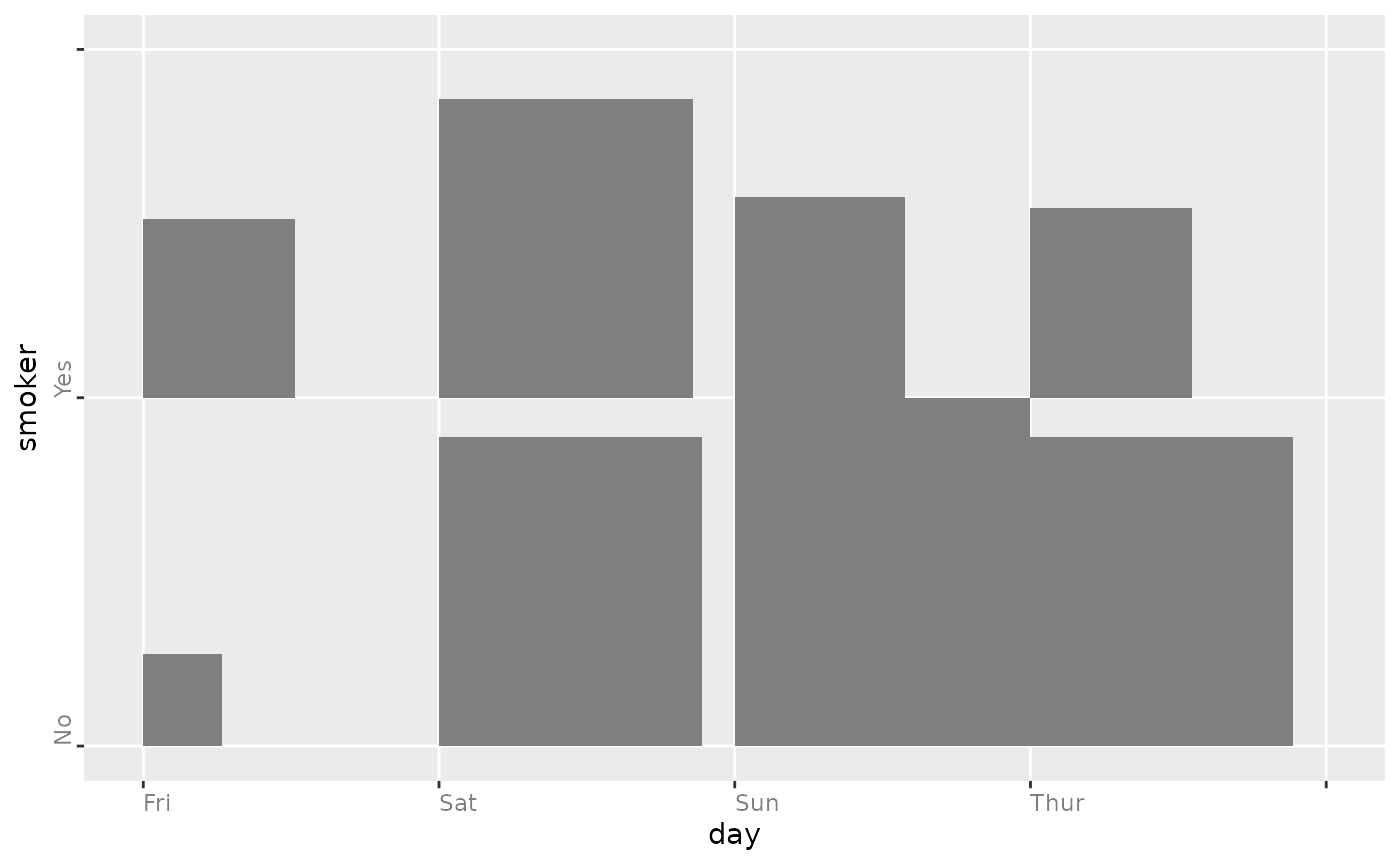ggally_ratio(tips, aes(x = day, y = smoker, colour = time))#### ggally_rowbar()

ggally_rowbar(tips, aes(x = day, y = smoker))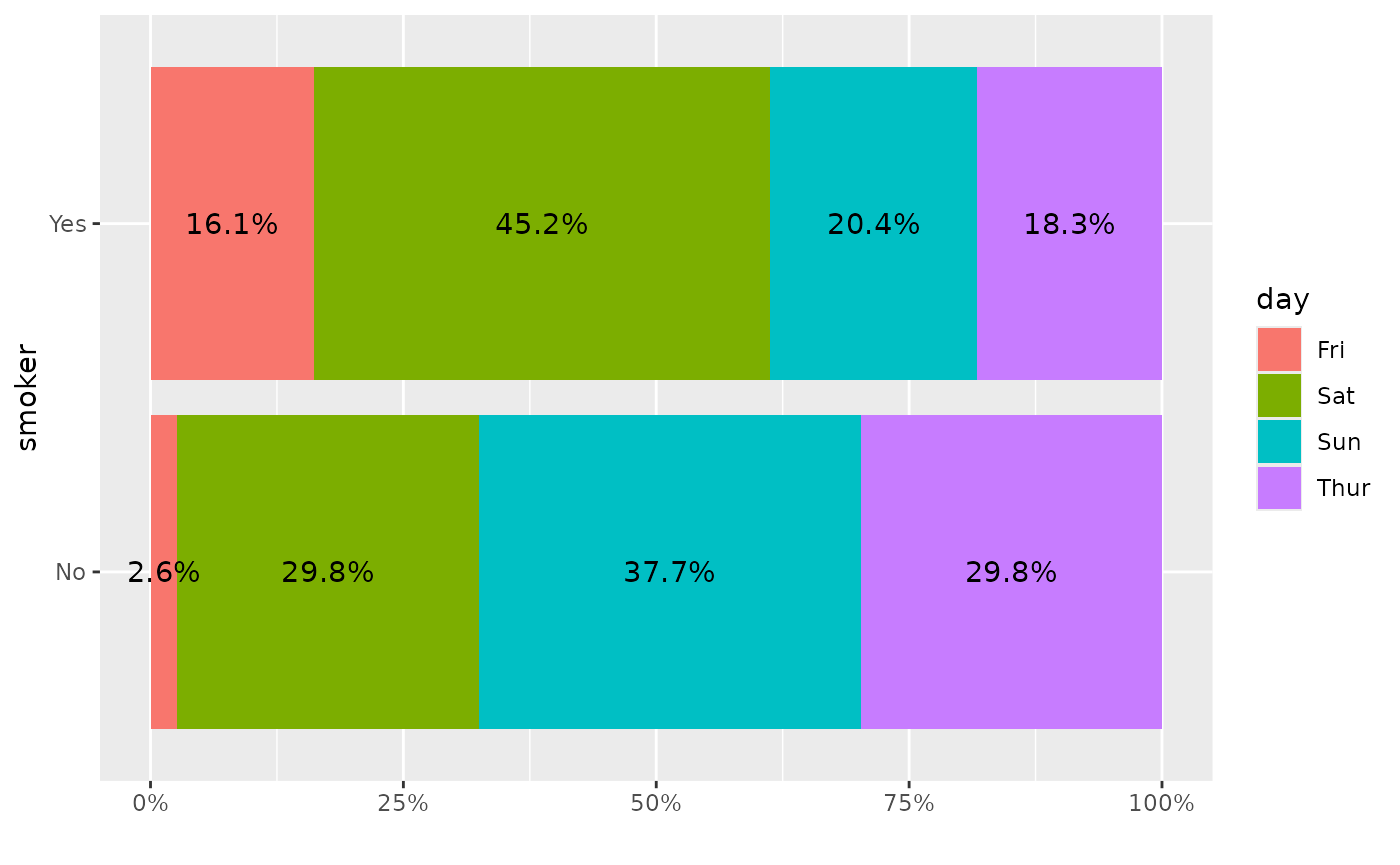Note: the colour aesthetic is not taken into account.

#### ggally_table()

ggally_table(tips, aes(x = day, y = smoker))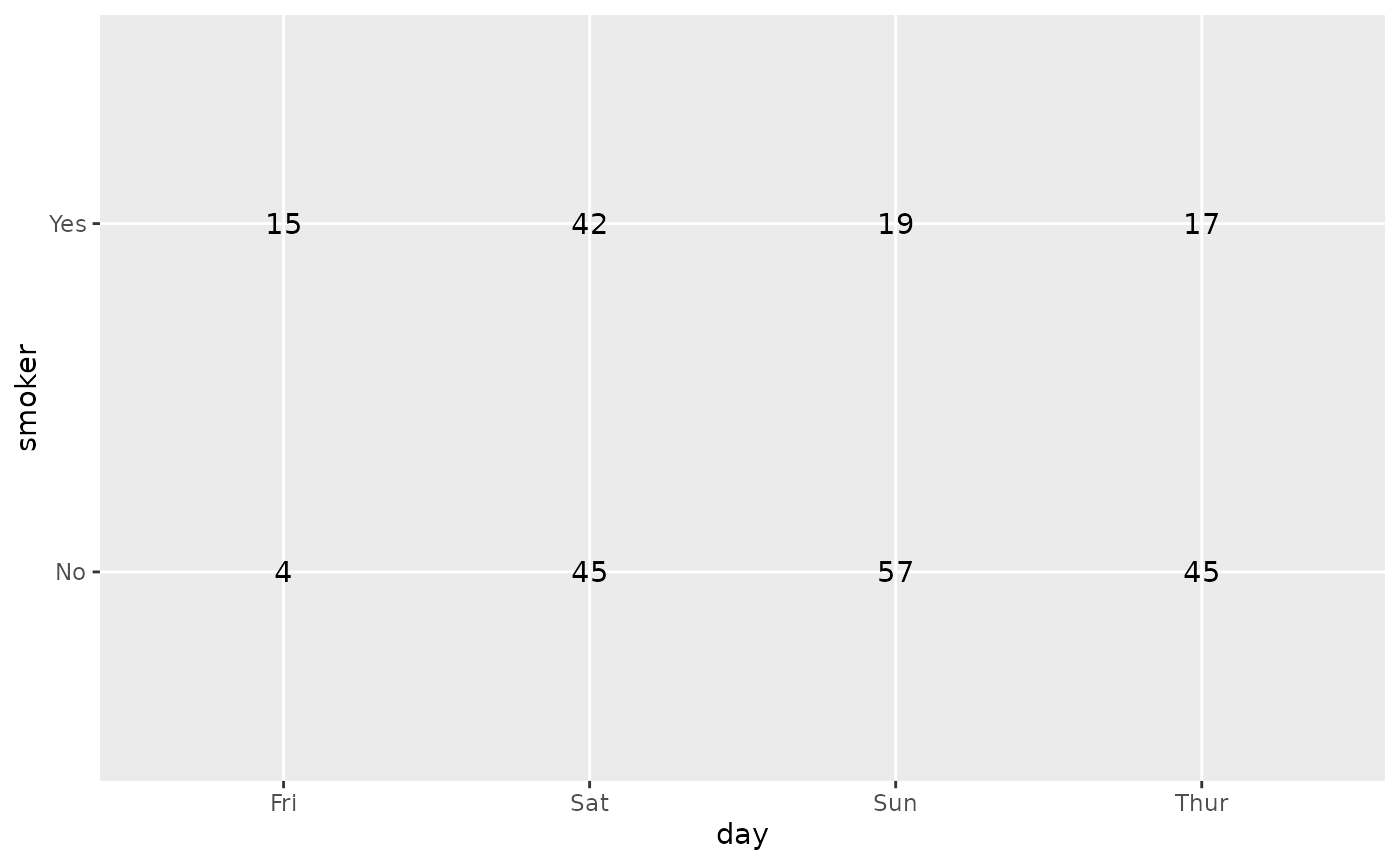ggally_table(tips, aes(x = day, y = smoker, colour = time))ggally_table(tips, aes(x = day, y = smoker, colour = smoker))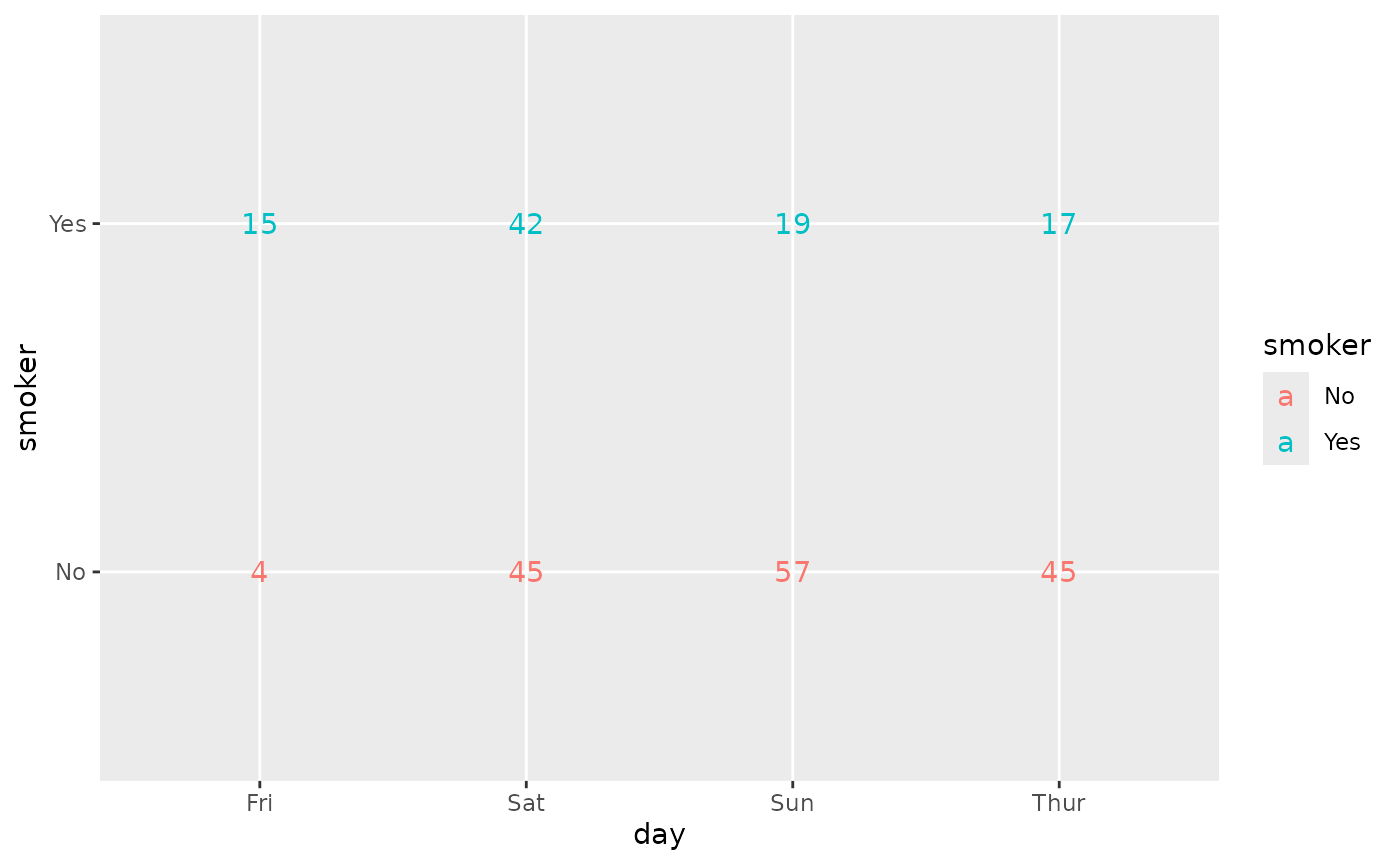Note: colour aesthetic is taken into account only if it corresponds to x or to y.

ggally_trends(tips, aes(x = day, y = smoker))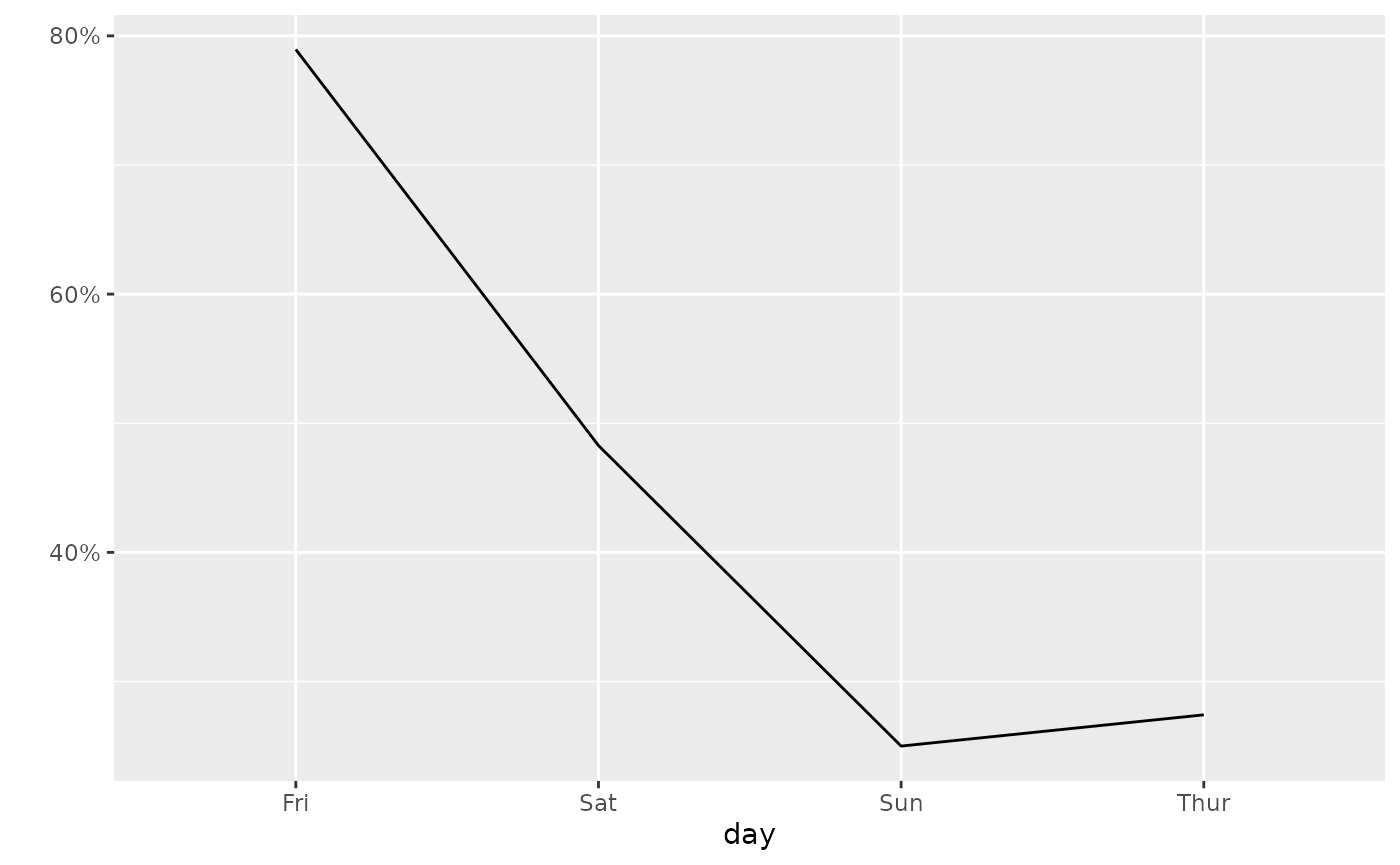ggally_trends(tips, aes(x = day, y = smoker, colour = time))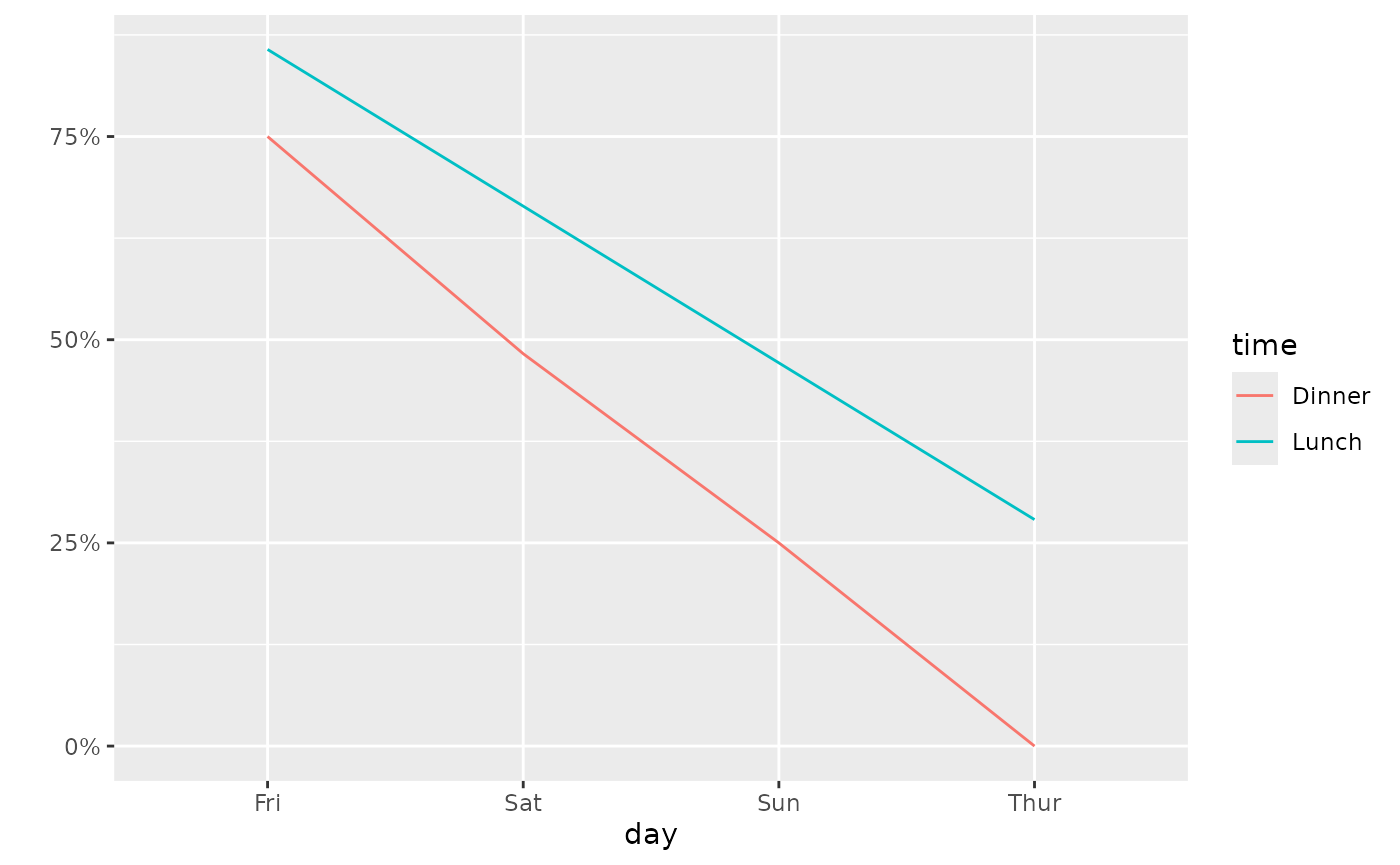### with 1x continuous and 1x discrete variables

#### ggally_autopoint()

ggally_autopoint(tips, aes(x = total_bill, y = day))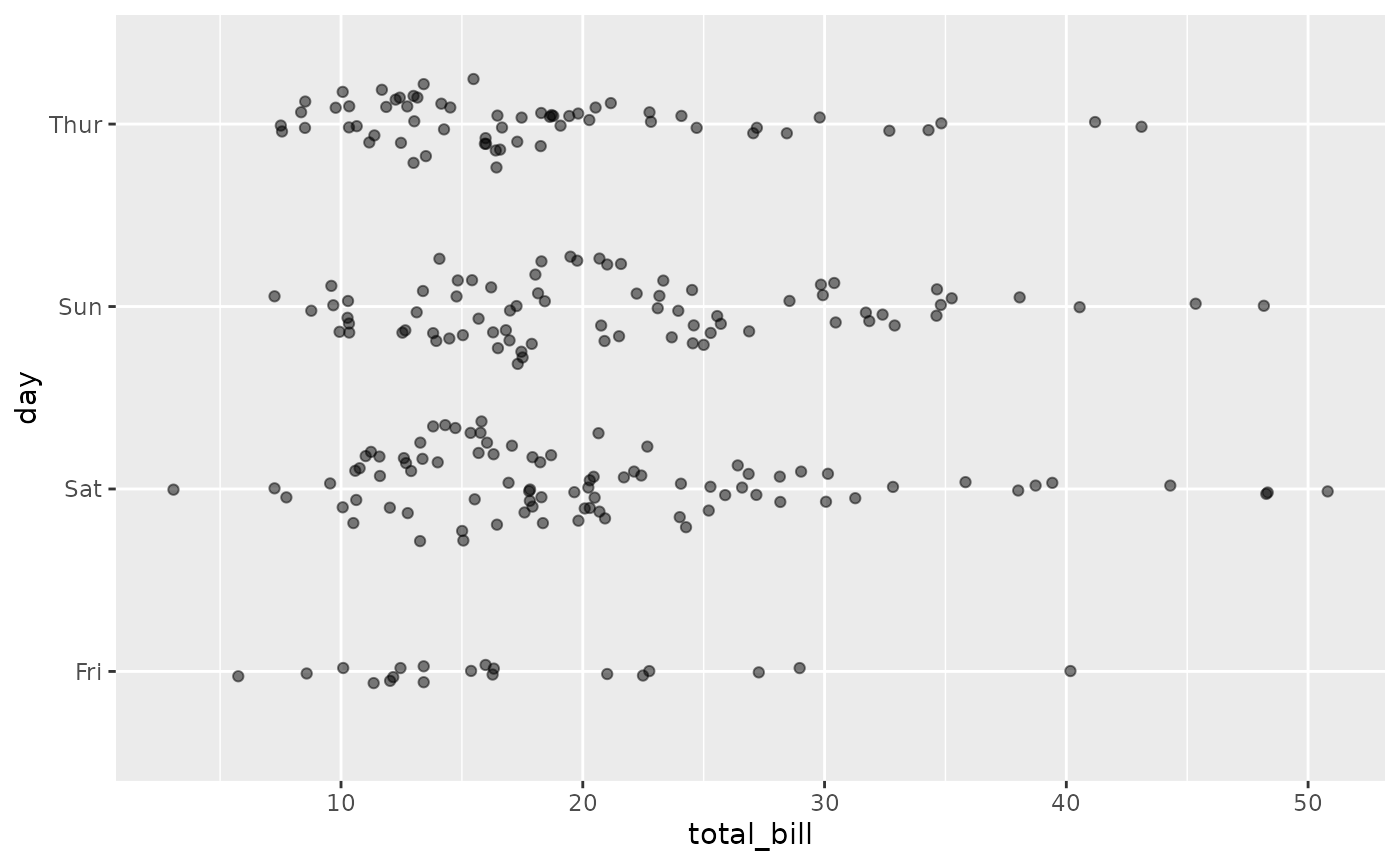ggally_autopoint(tips, aes(x = total_bill, y = day, colour = time))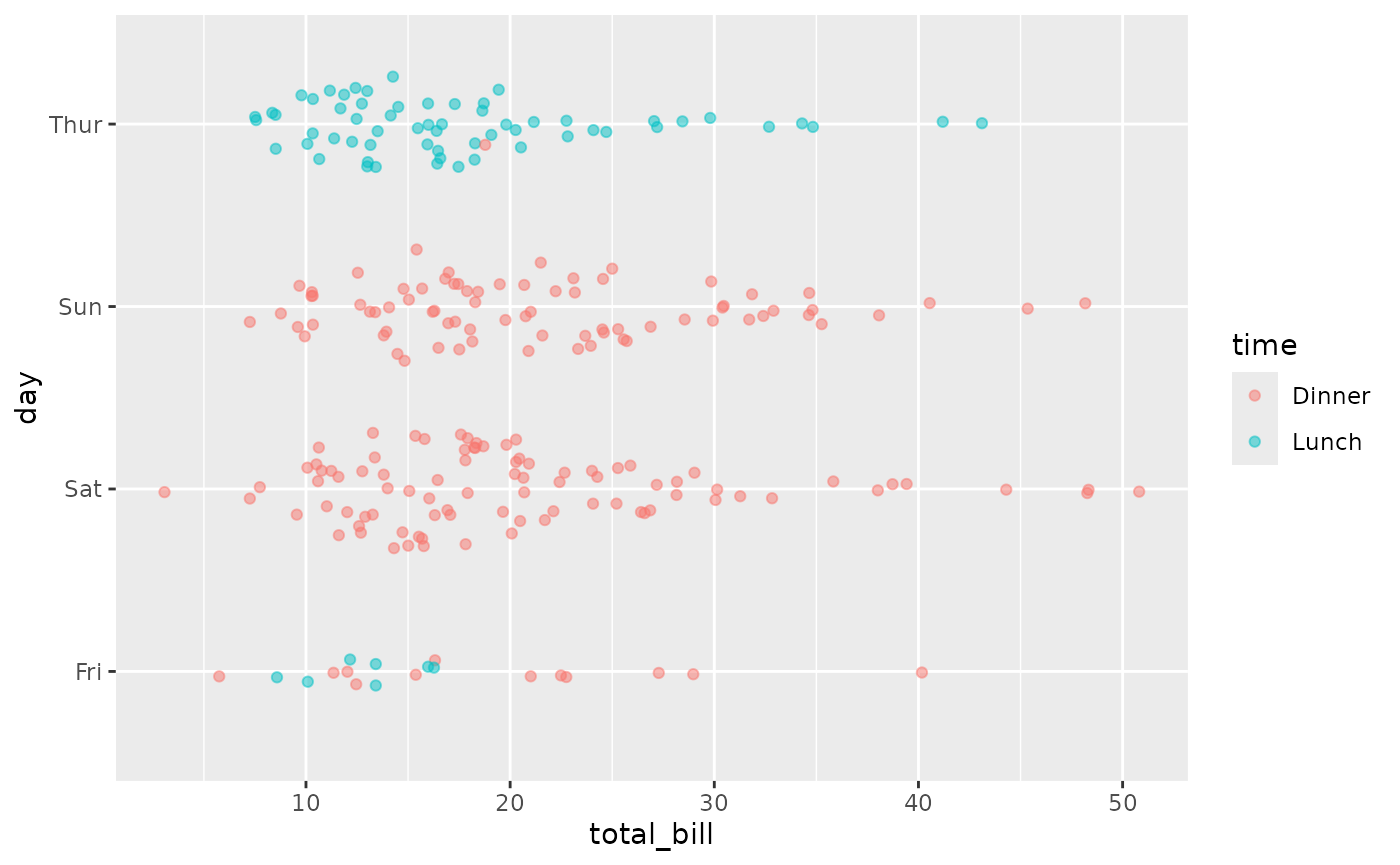#### ggally_box() & ggally_box_no_facet()

ggally_box(tips, aes(x = total_bill, y = day))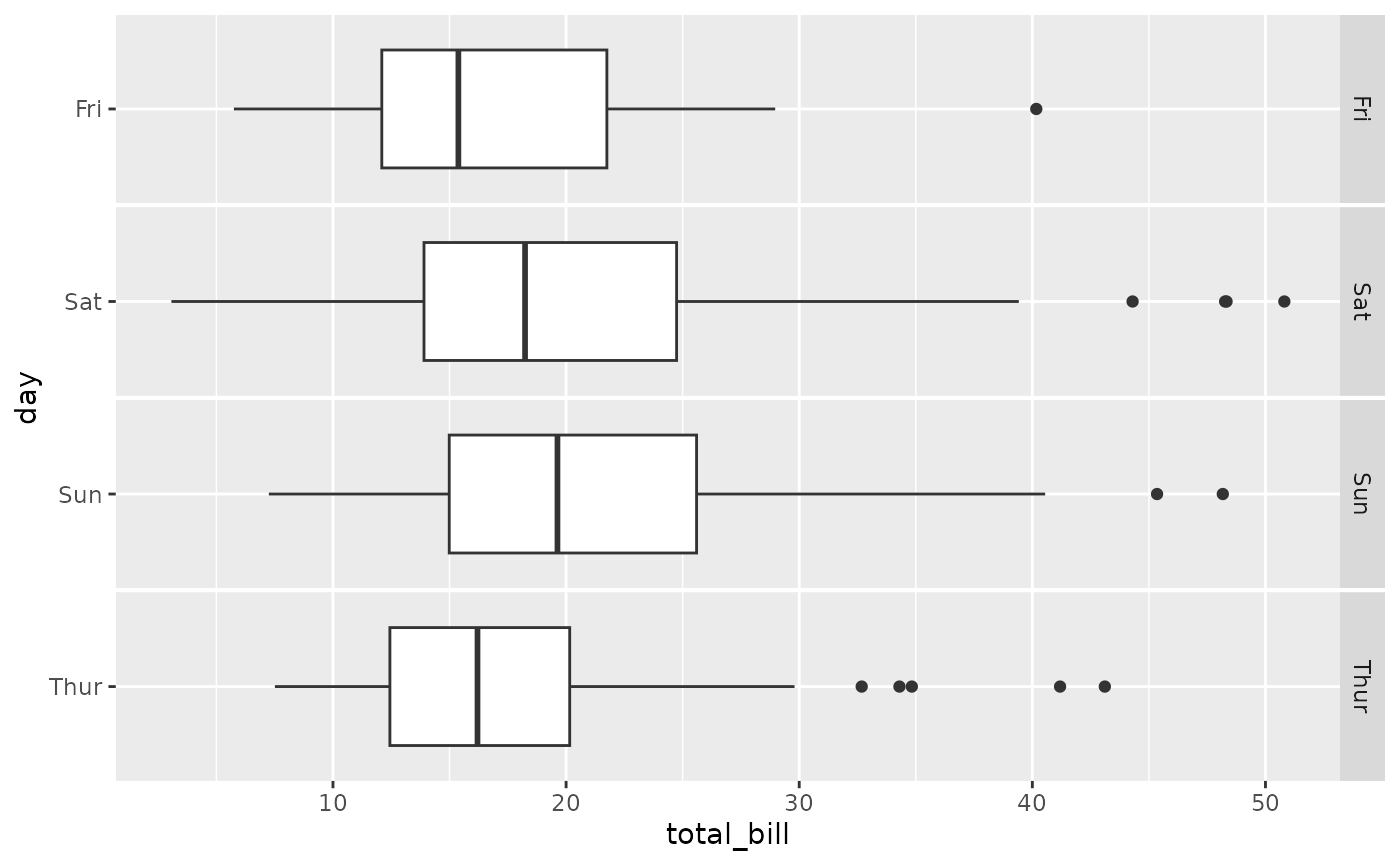ggally_box(tips, aes(x = total_bill, y = day, colour = time))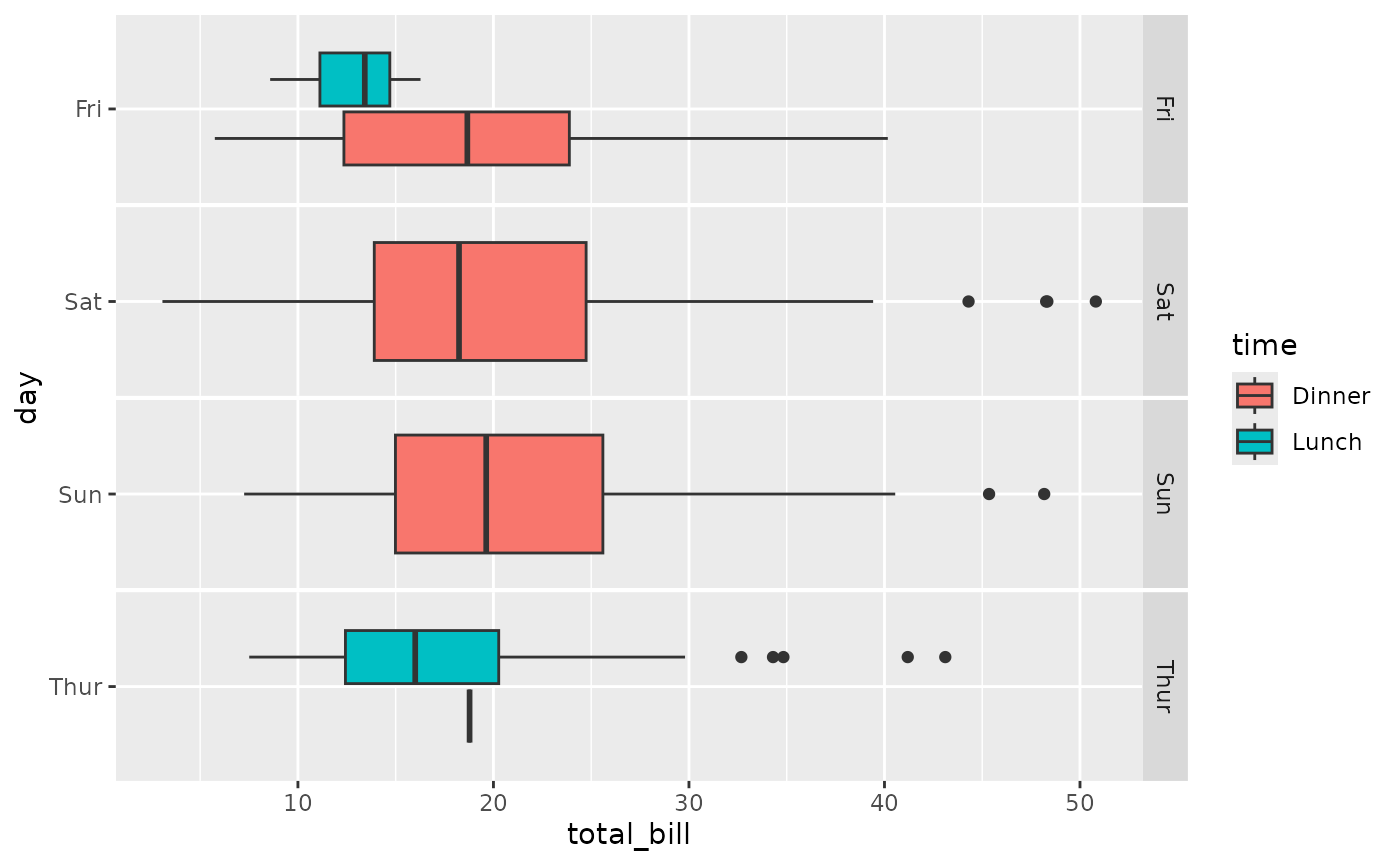ggally_box_no_facet(tips, aes(x = total_bill, y = day))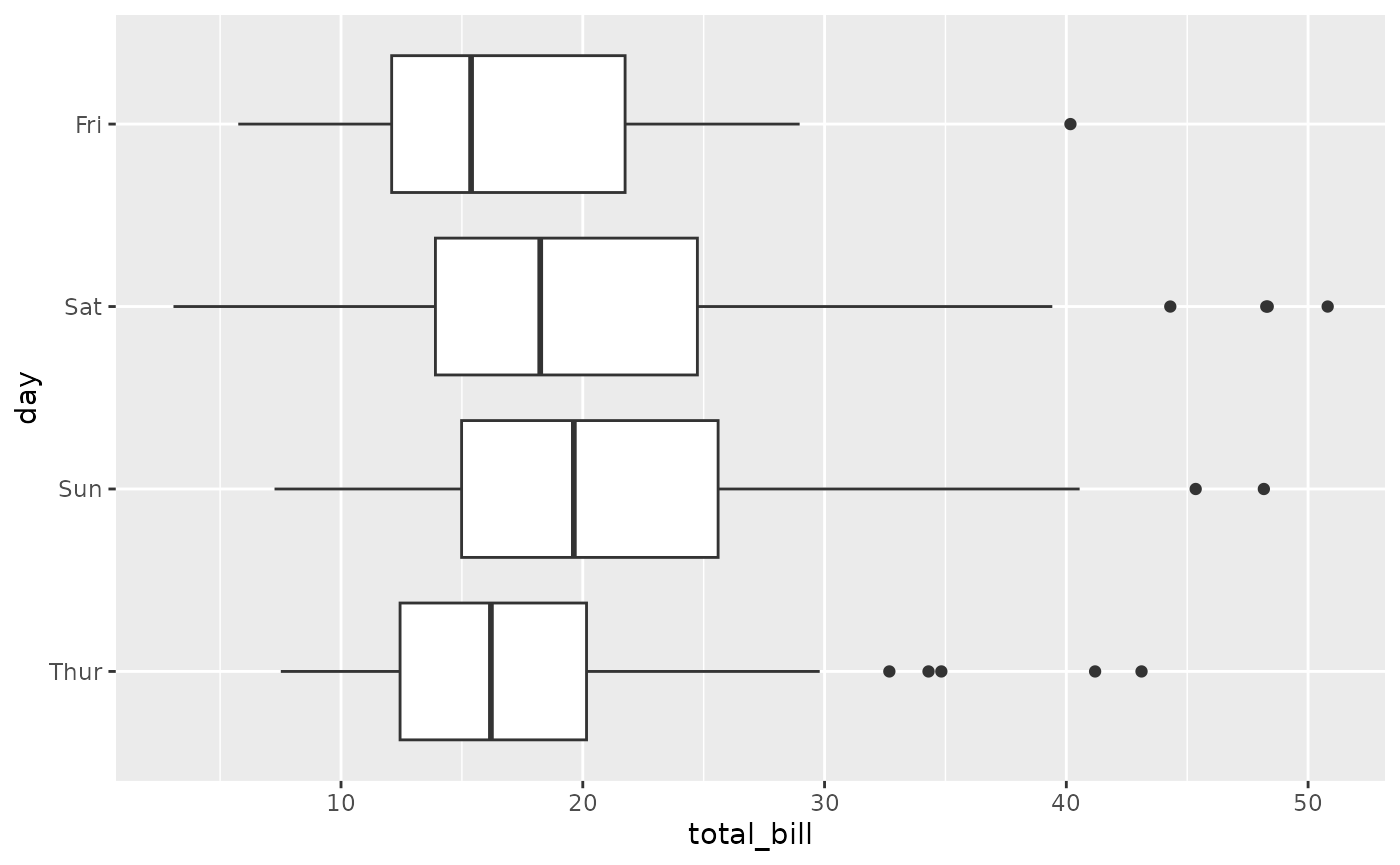ggally_box_no_facet(tips, aes(x = total_bill, y = day, colour = time))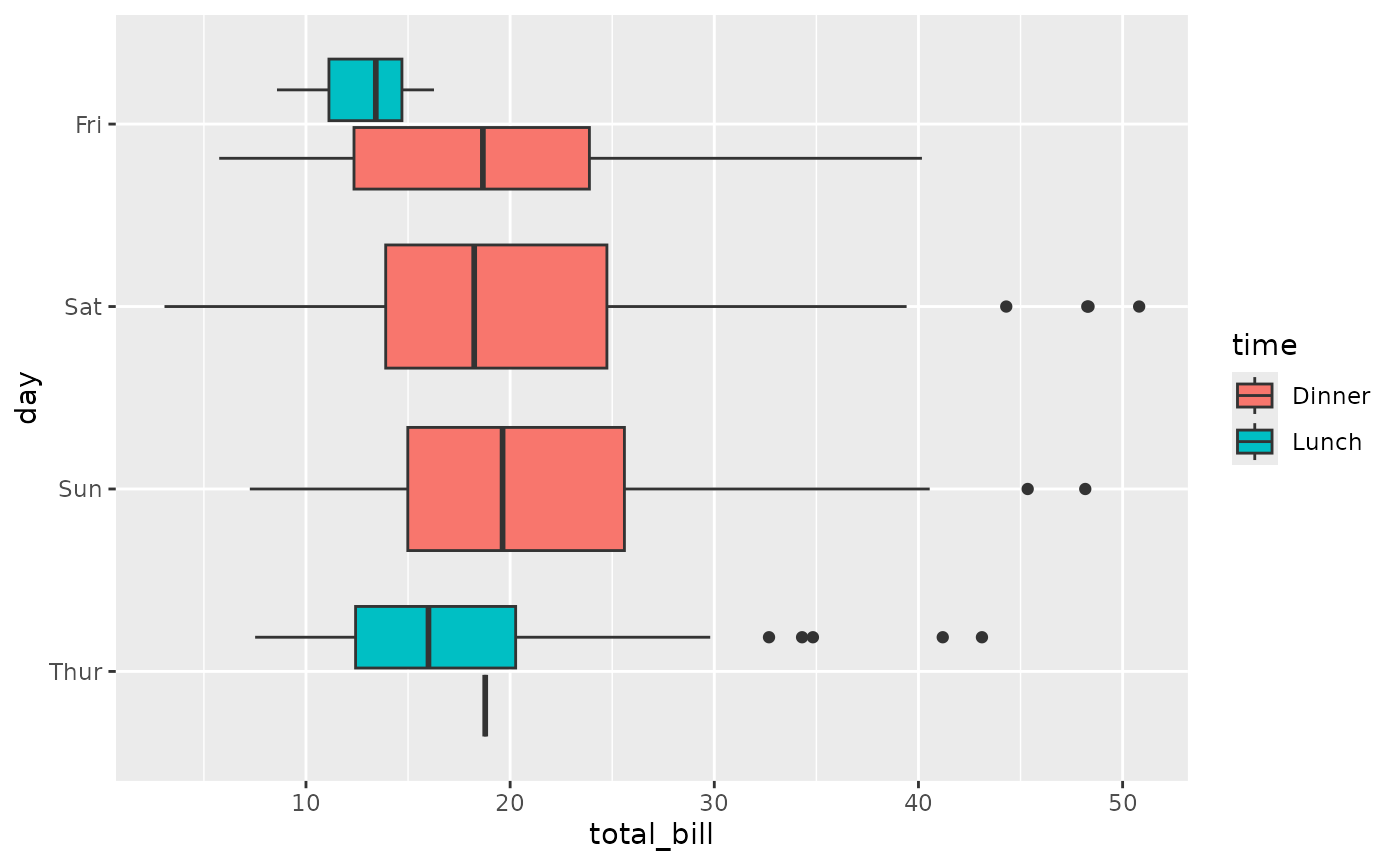#### ggally_denstrip()

ggally_denstrip(tips, aes(x = total_bill, y = day))
#> stat_bin() using bins = 30. Pick better value with binwidth.
#> Warning: Removed 45 rows containing missing values (geom_bar()).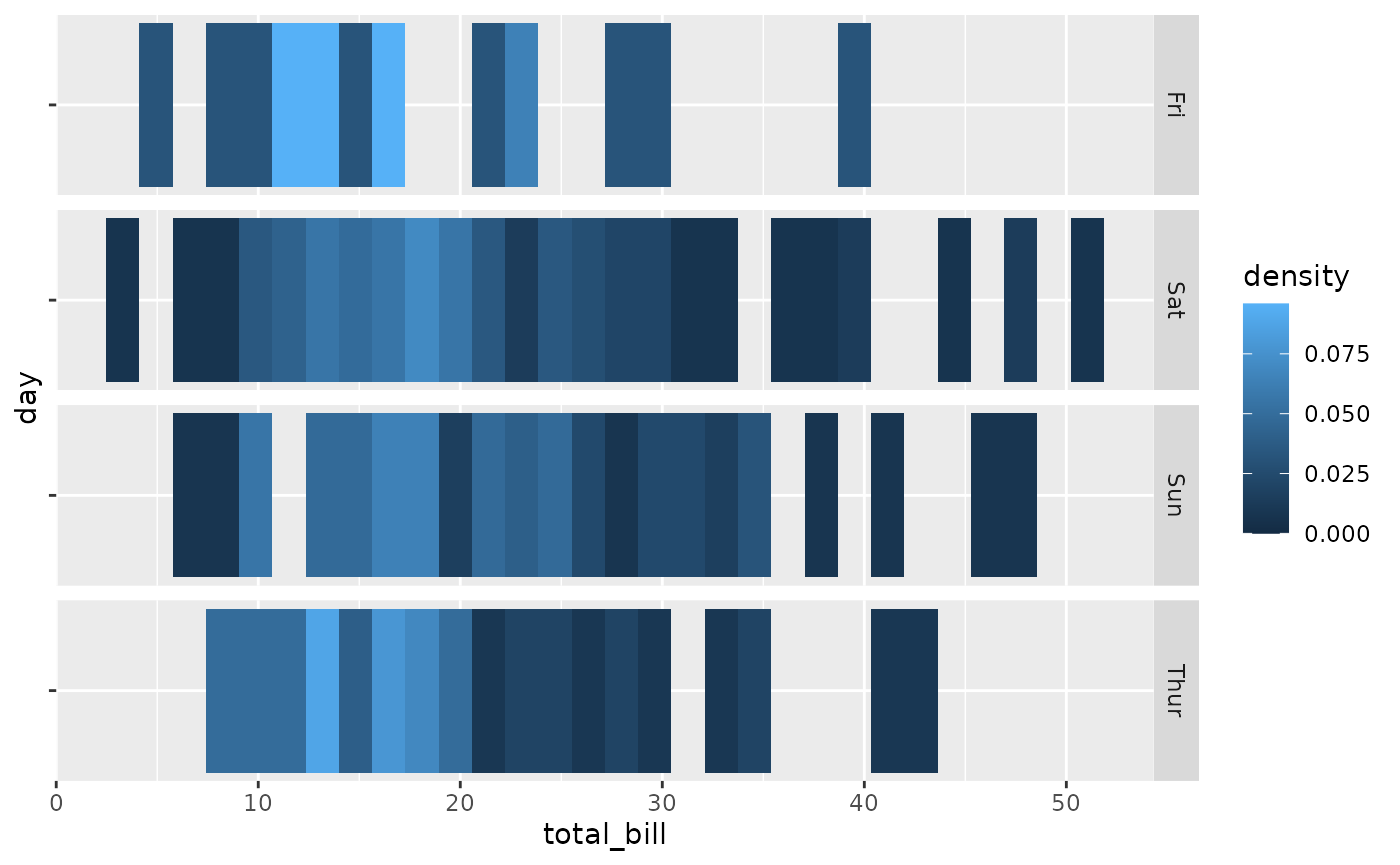ggally_denstrip(tips, aes(x = total_bill, y = day, colour = time))
#> stat_bin() using bins = 30. Pick better value with binwidth.
#> Warning: Removed 45 rows containing missing values (geom_bar()).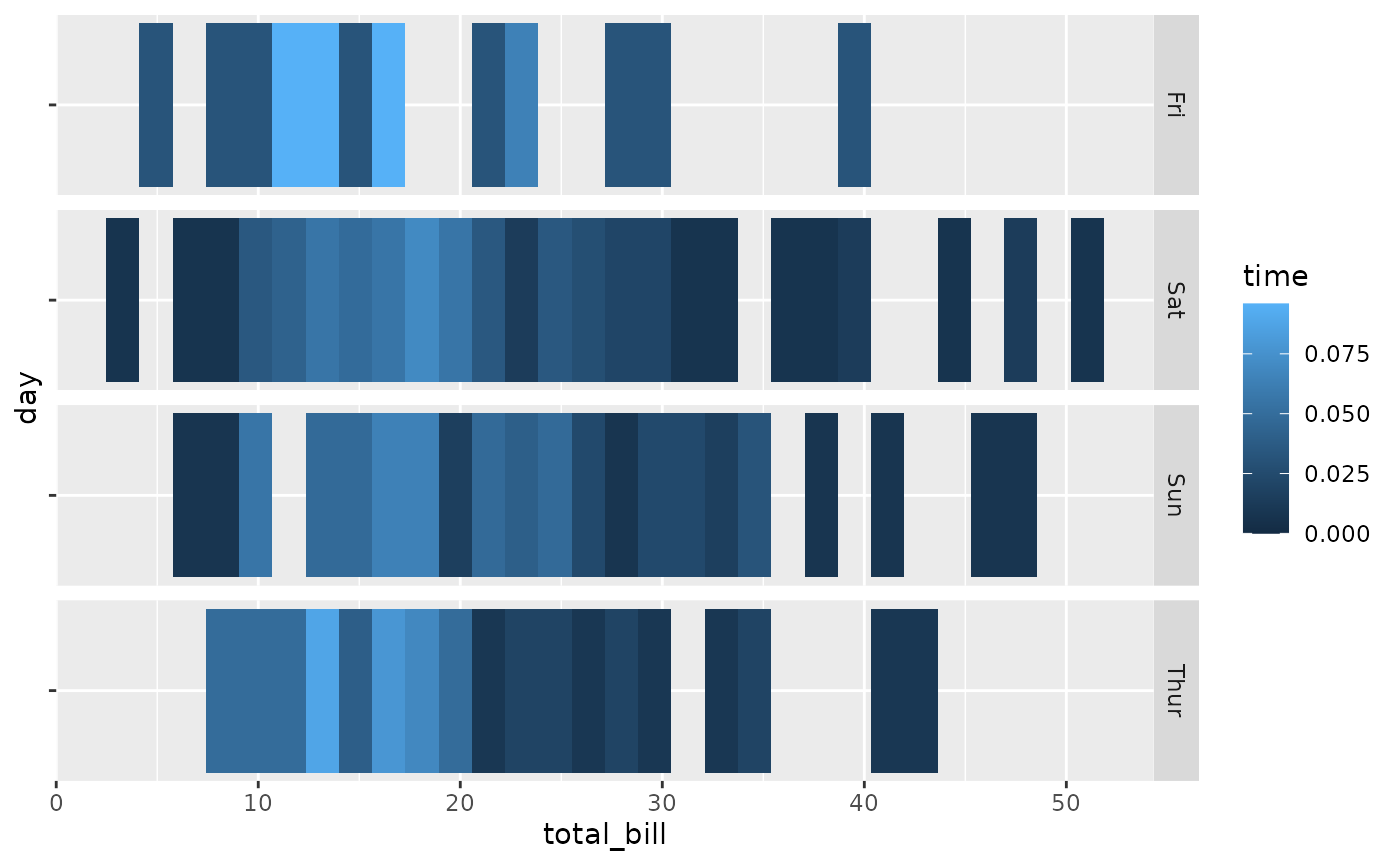#### ggally_dot() & ggally_dot_no_facet()

ggally_dot(tips, aes(x = total_bill, y = day))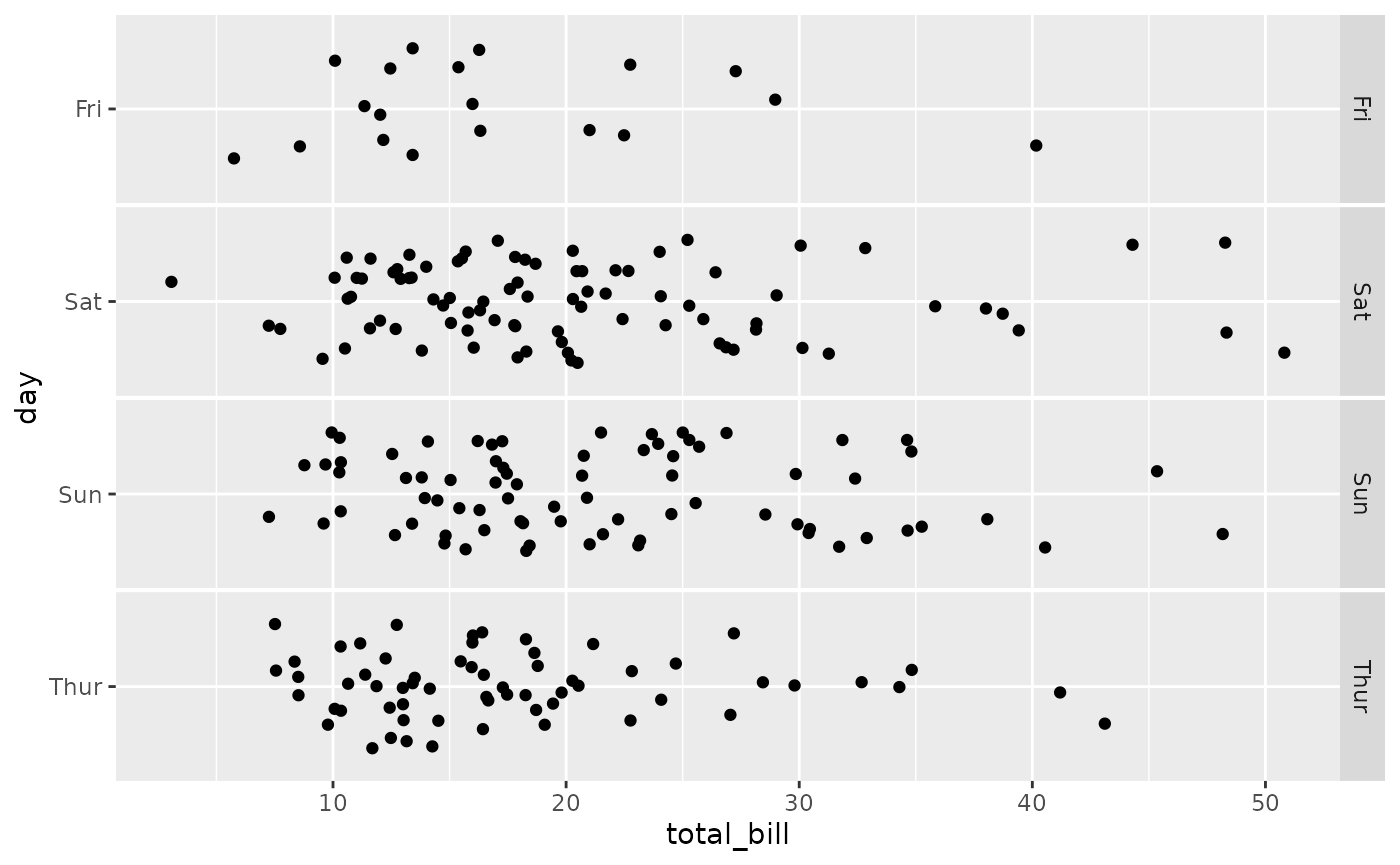ggally_dot(tips, aes(x = total_bill, y = day, colour = time))ggally_dot_no_facet(tips, aes(x = total_bill, y = day))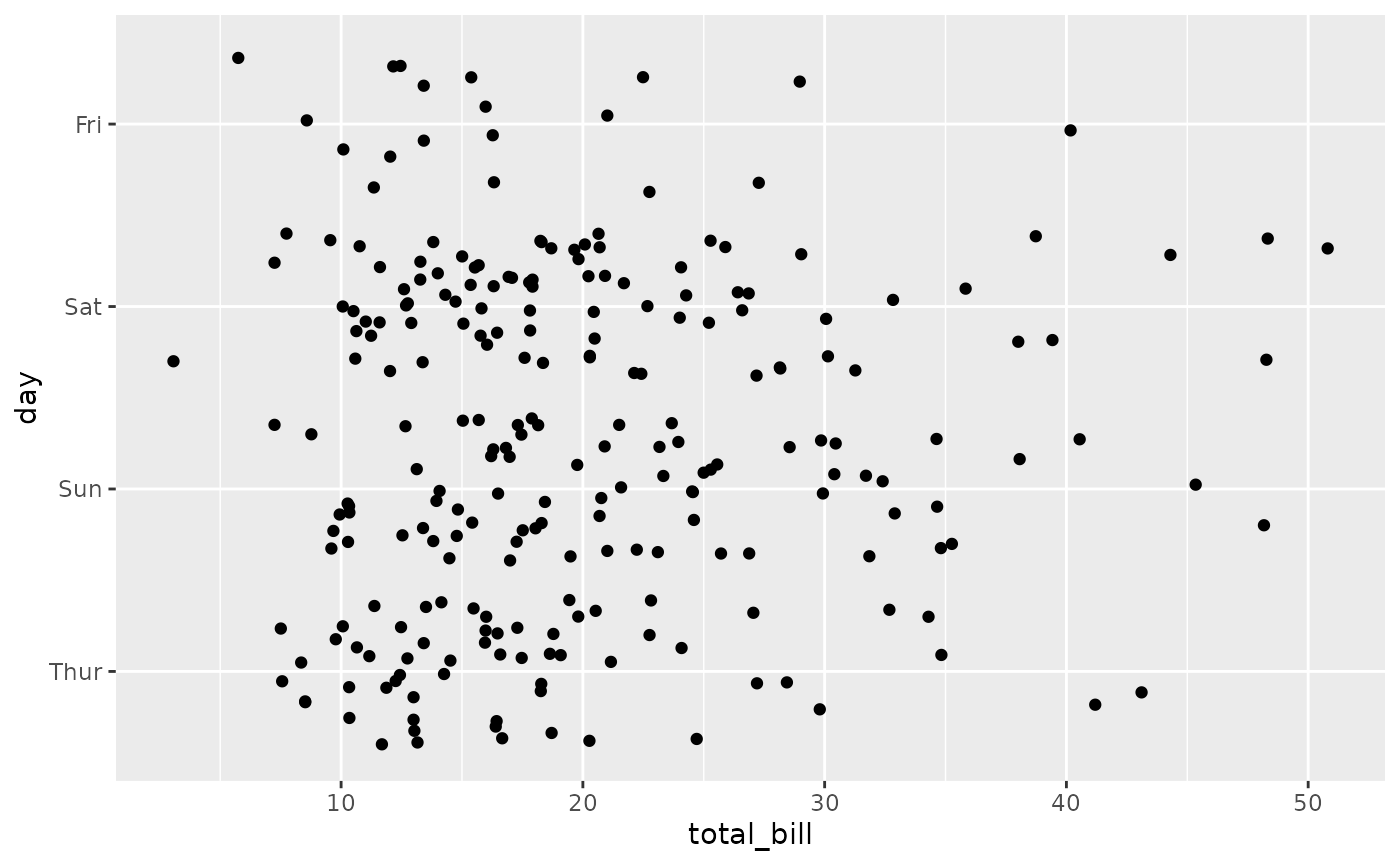ggally_dot_no_facet(tips, aes(x = total_bill, y = day, colour = time))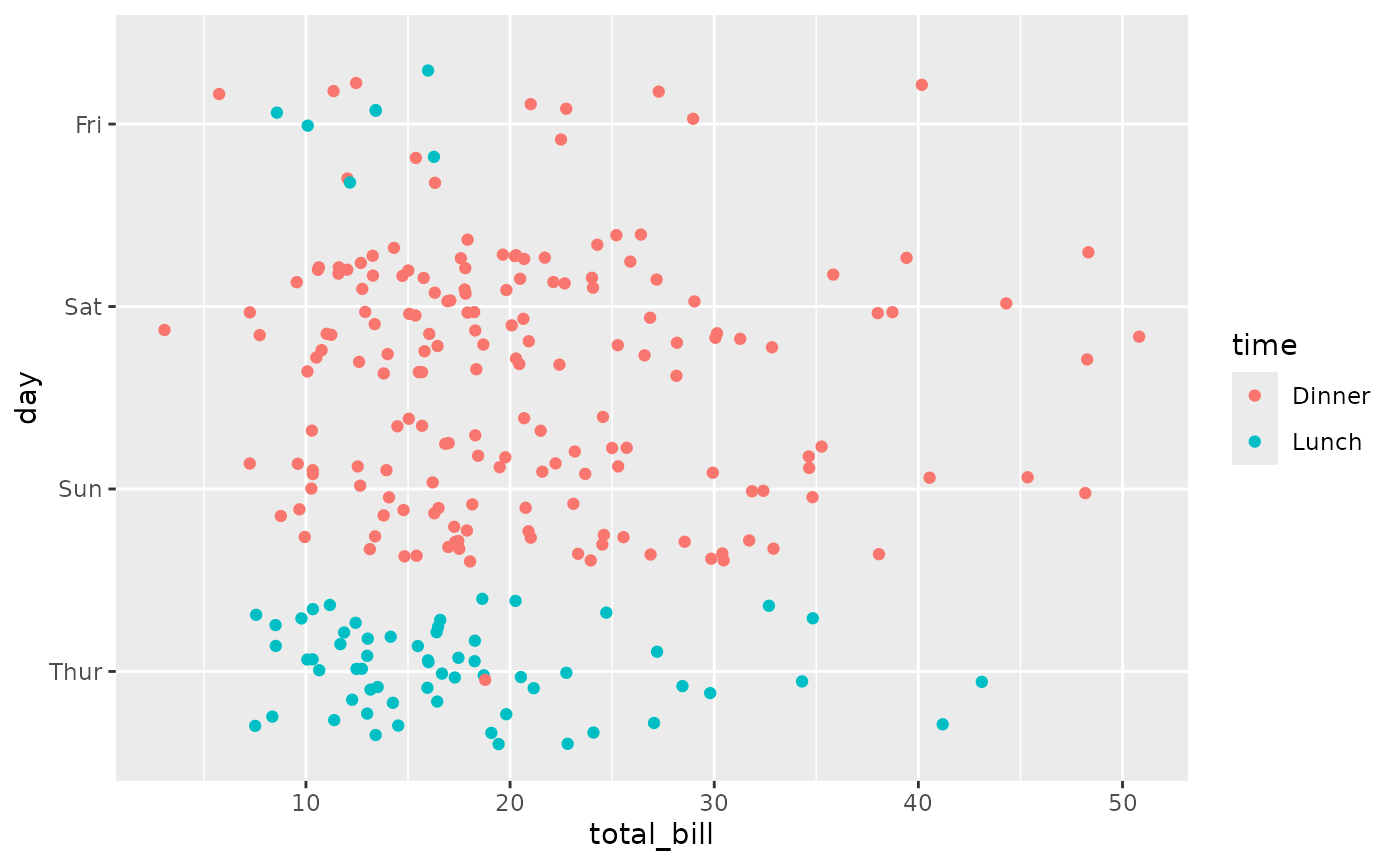#### ggally_facetdensitystrip()

ggally_facetdensitystrip(tips, aes(x = total_bill, y = day))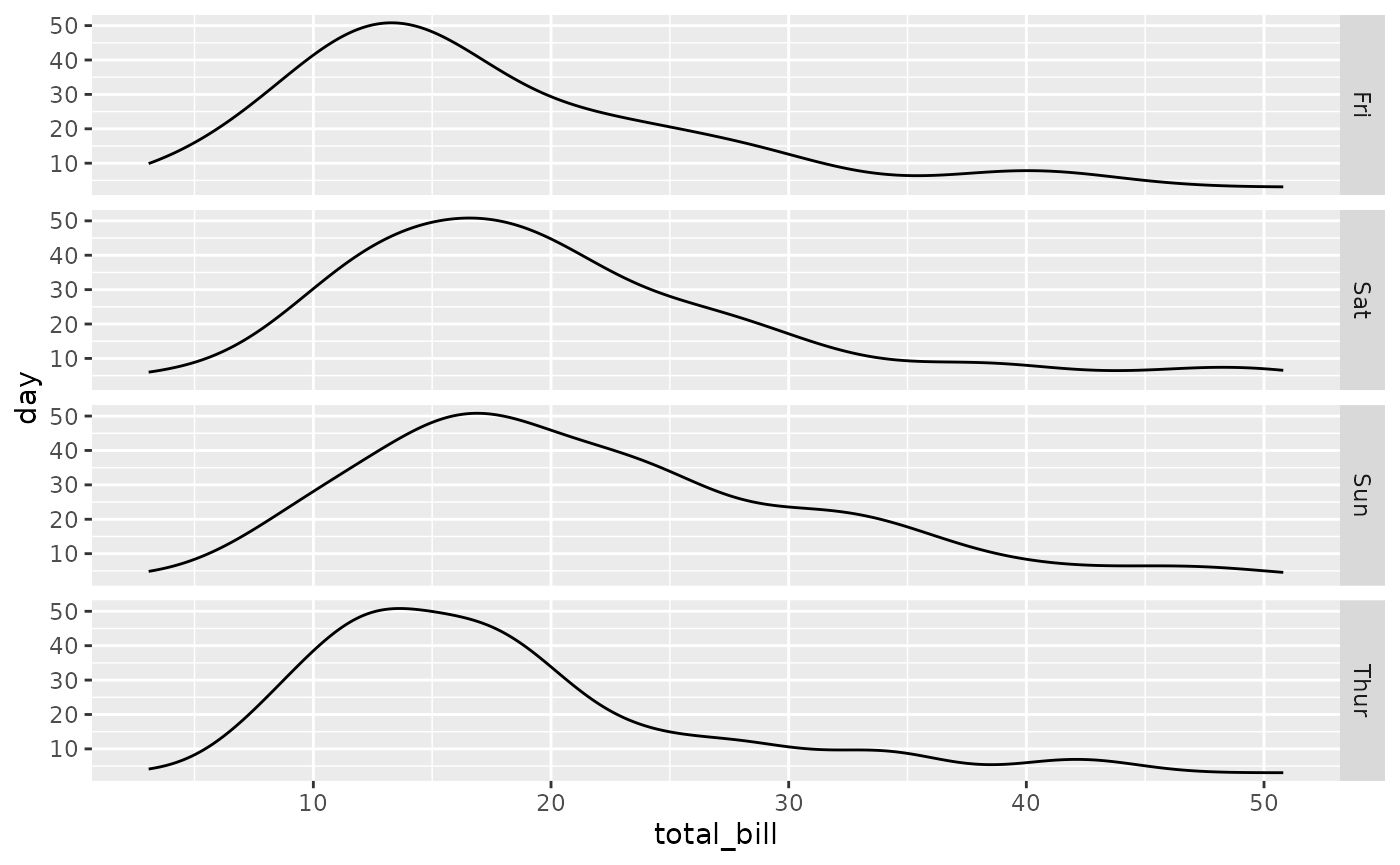ggally_facetdensitystrip(tips, aes(x = total_bill, y = day, colour = time))
#> Warning: Groups with fewer than two data points have been dropped.
#> Warning: Removed 1 row containing missing values (geom_line()).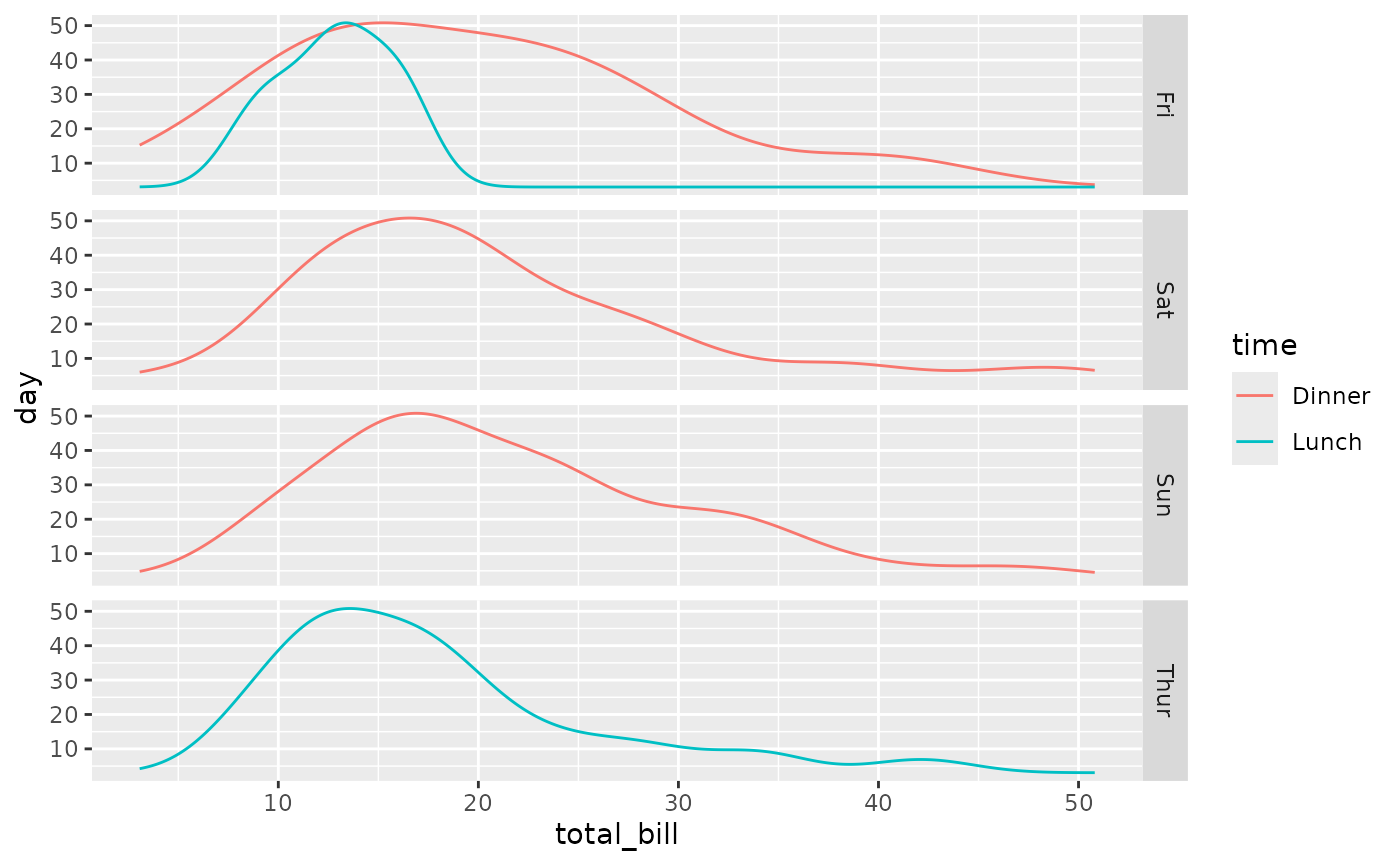#### ggally_facethist()

ggally_facethist(tips, aes(x = total_bill, y = day))
#> stat_bin() using bins = 30. Pick better value with binwidth.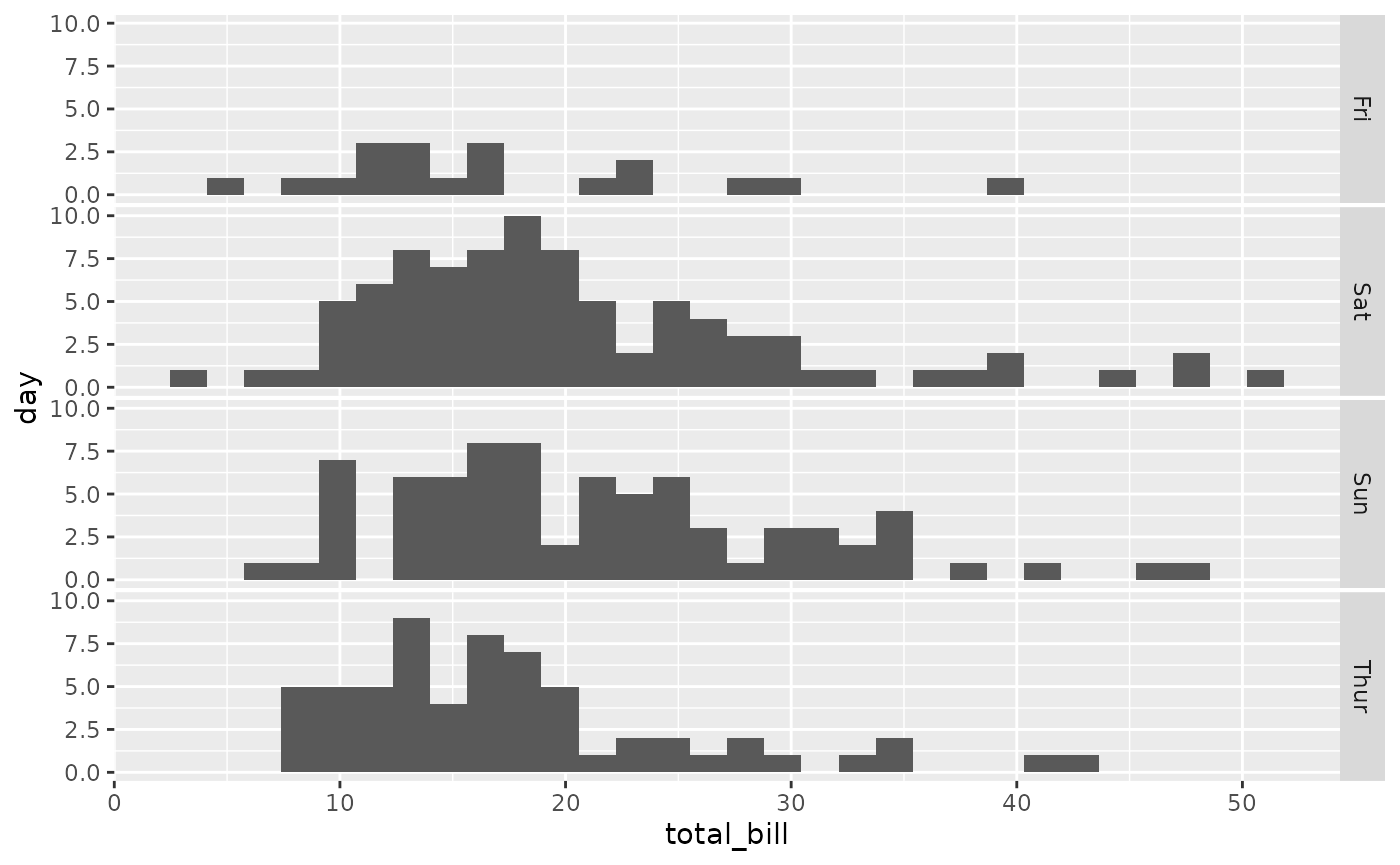ggally_facethist(tips, aes(x = total_bill, y = day, colour = time))
#> stat_bin() using bins = 30. Pick better value with binwidth.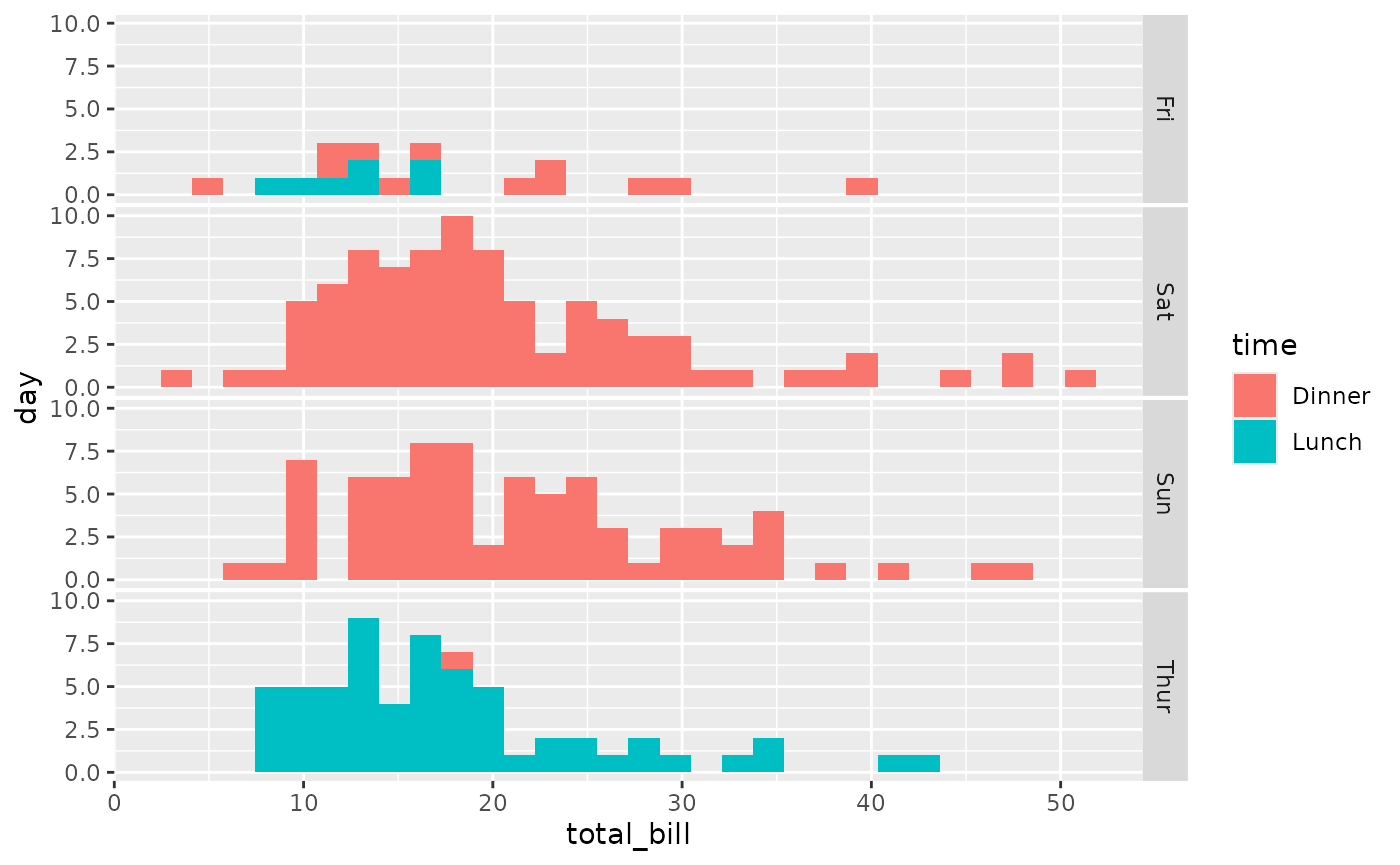#### ggally_summarise_by()

ggally_summarise_by(tips, aes(x = total_bill, y = day))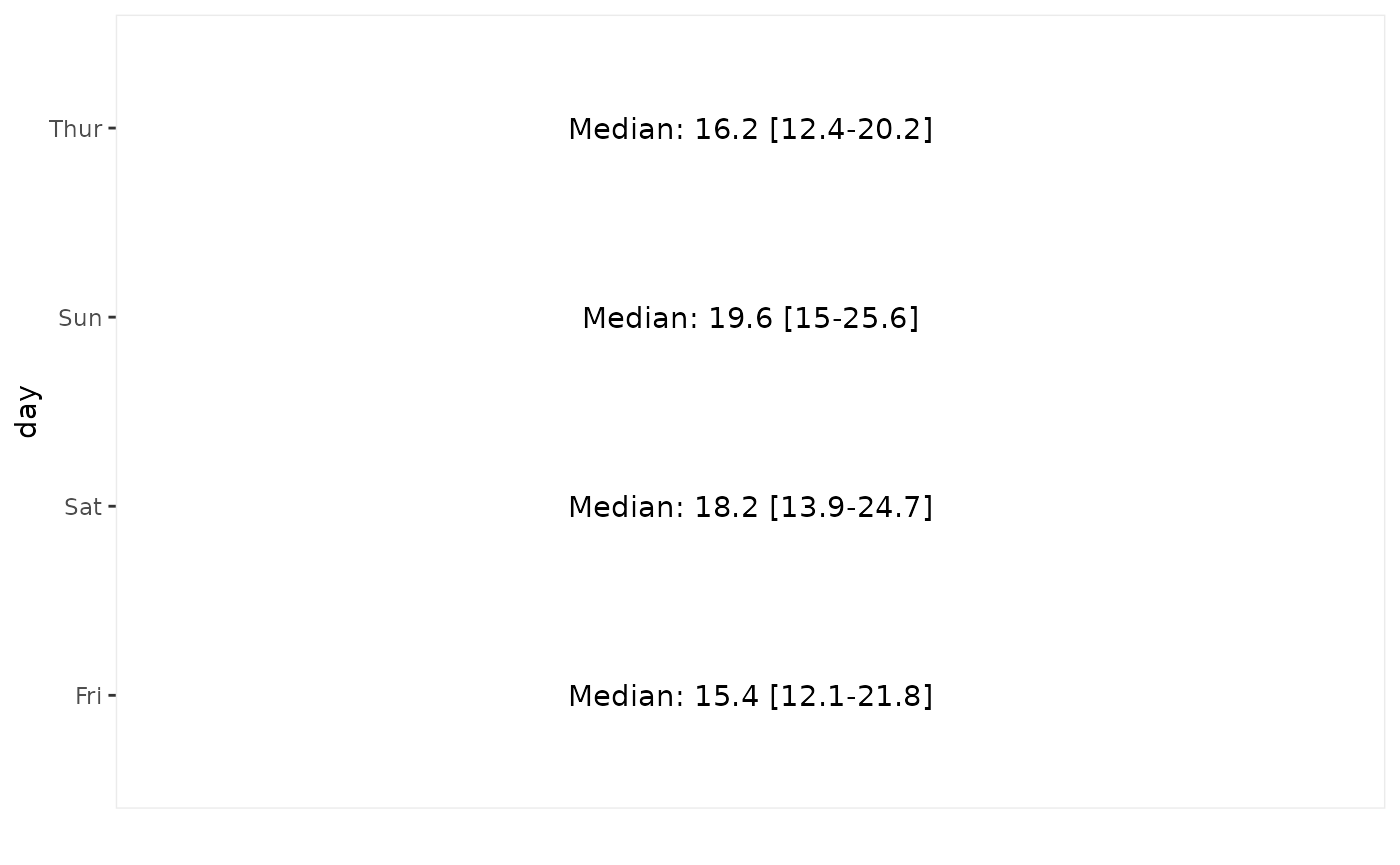ggally_summarise_by(tips, aes(x = total_bill, y = day, colour = day))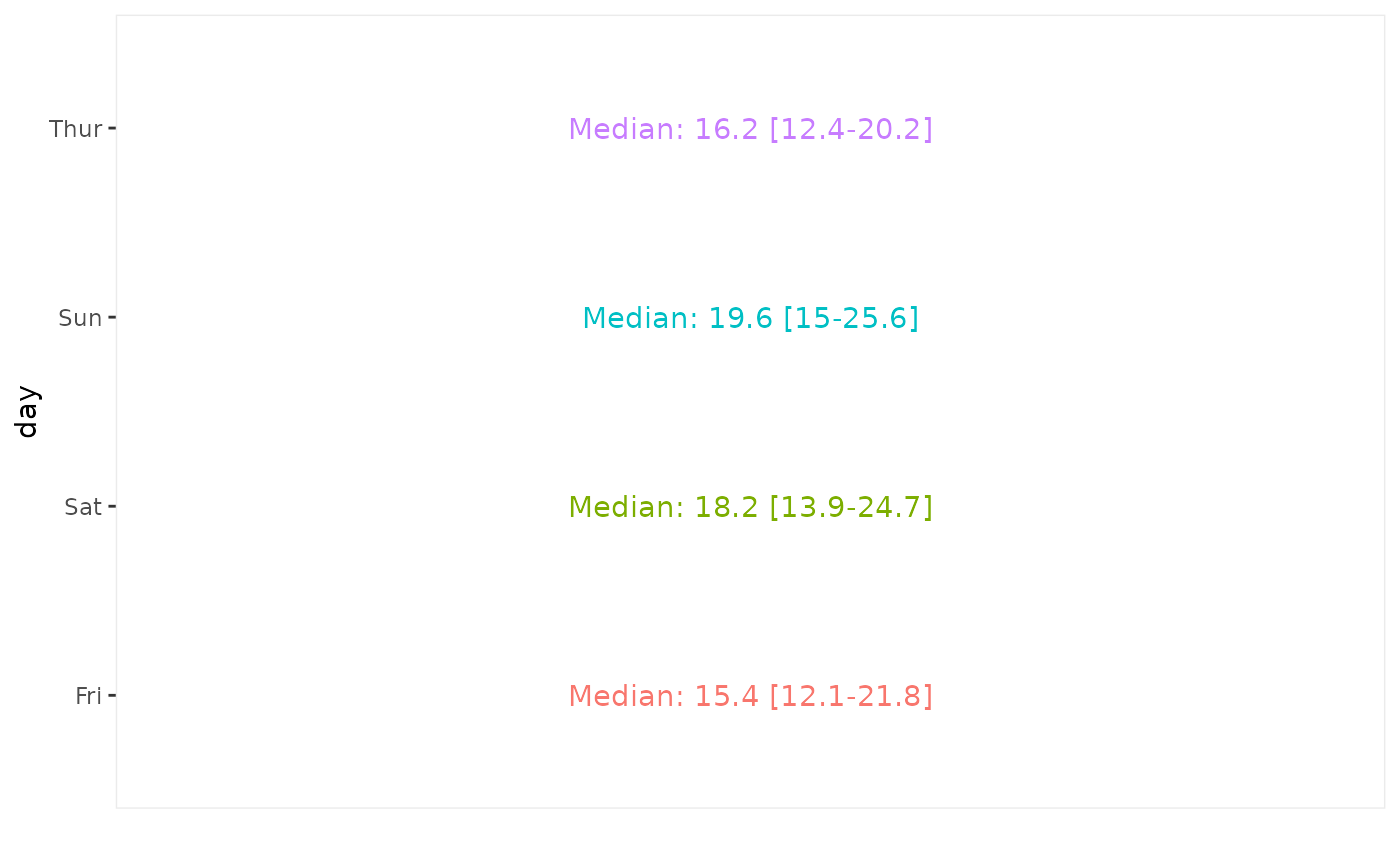Note: colour aesthetic is kept only if corresponding to the discrete axis.

ggally_trends(tips, aes(y = total_bill, x = day))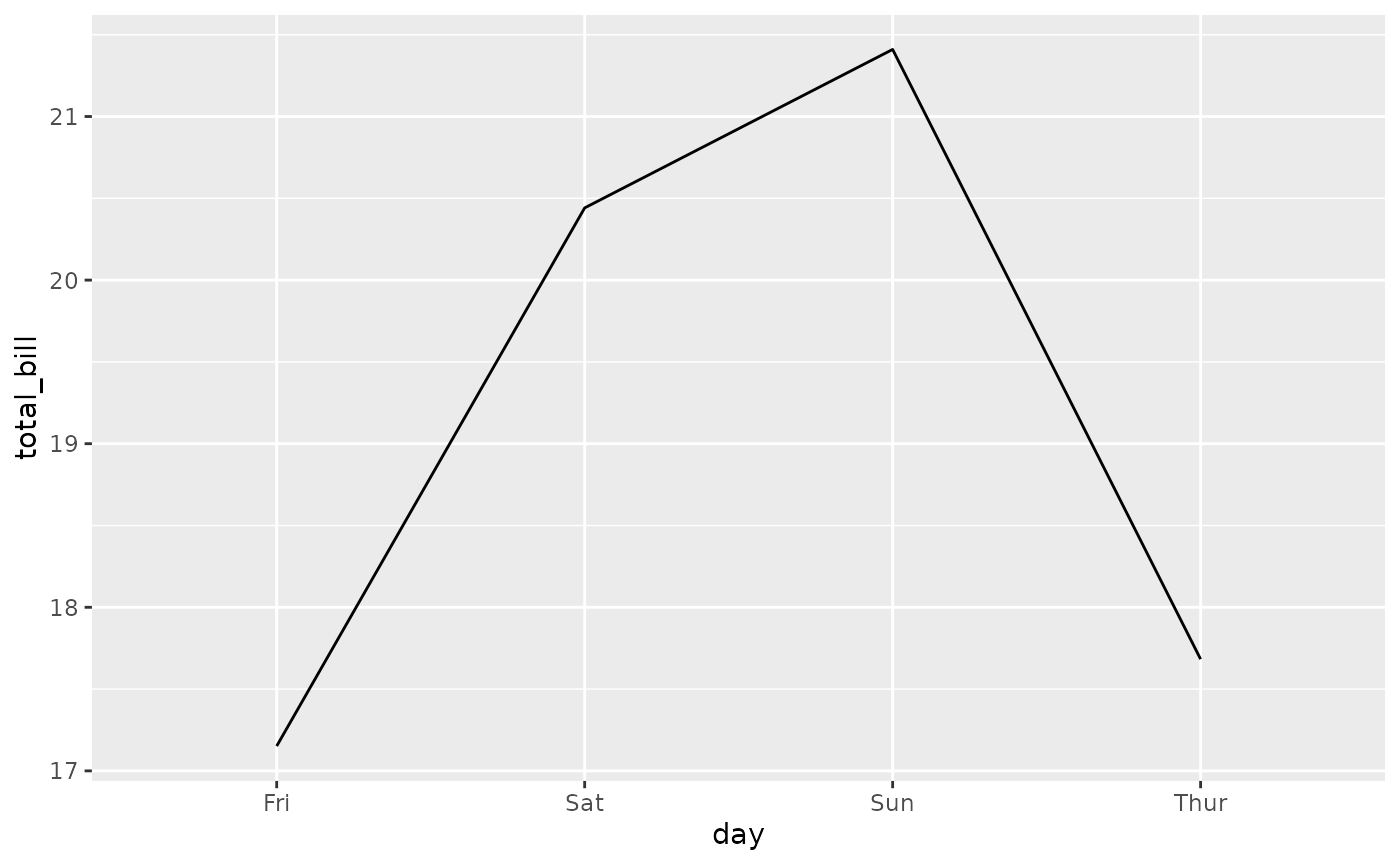ggally_trends(tips, aes(y = total_bill, x = day, colour = time))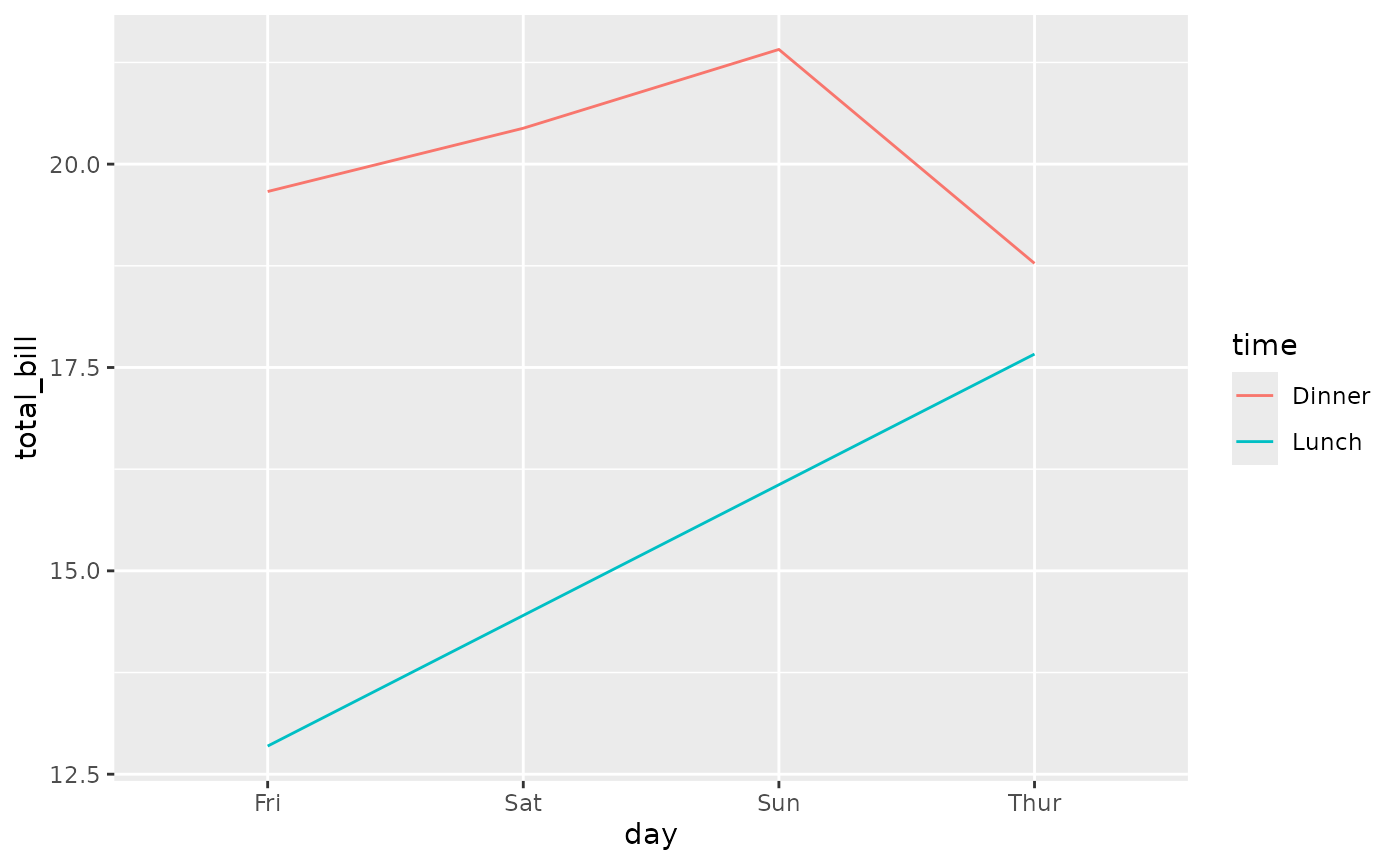## Diagonal plots

### with 1x continuous variable

#### ggally_autopointDiag()

ggally_autopointDiag(tips, aes(x = total_bill))ggally_autopointDiag(tips, aes(x = total_bill, colour = time))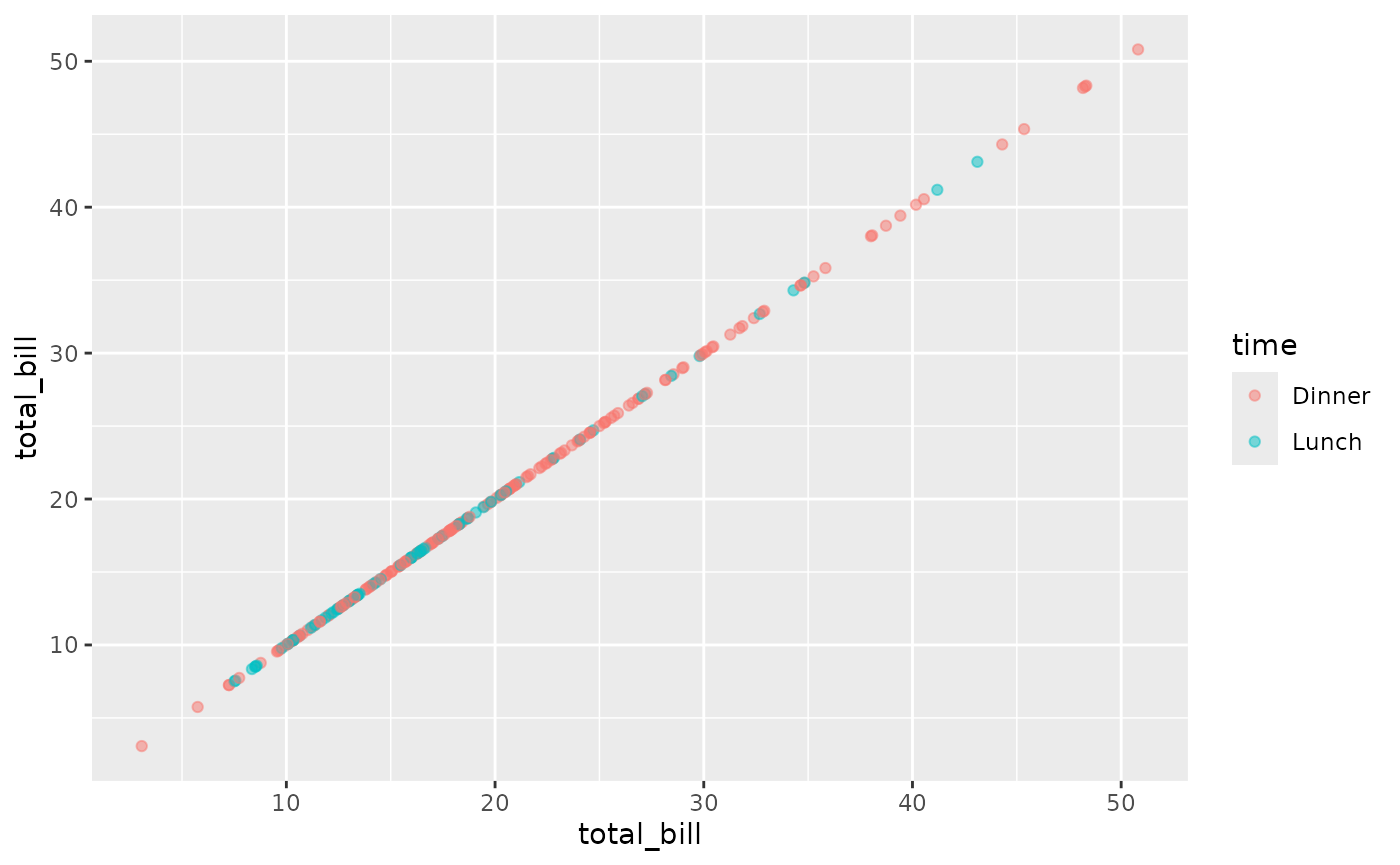#### ggally_barDiag()

ggally_barDiag(tips, aes(x = total_bill))
#> stat_bin() using bins = 30. Pick better value with binwidth.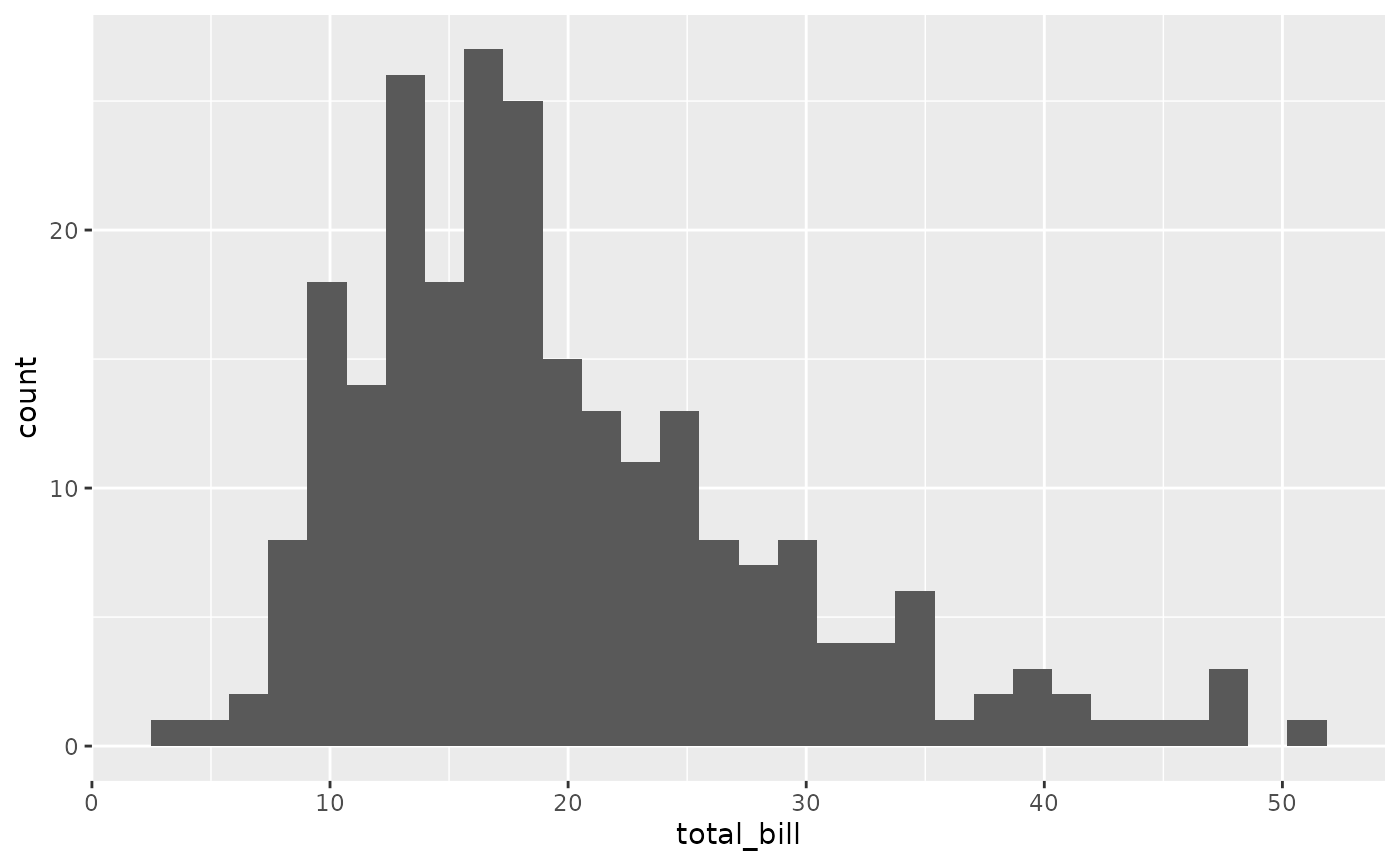ggally_barDiag(tips, aes(x = total_bill, colour = time))
#> stat_bin() using bins = 30. Pick better value with binwidth.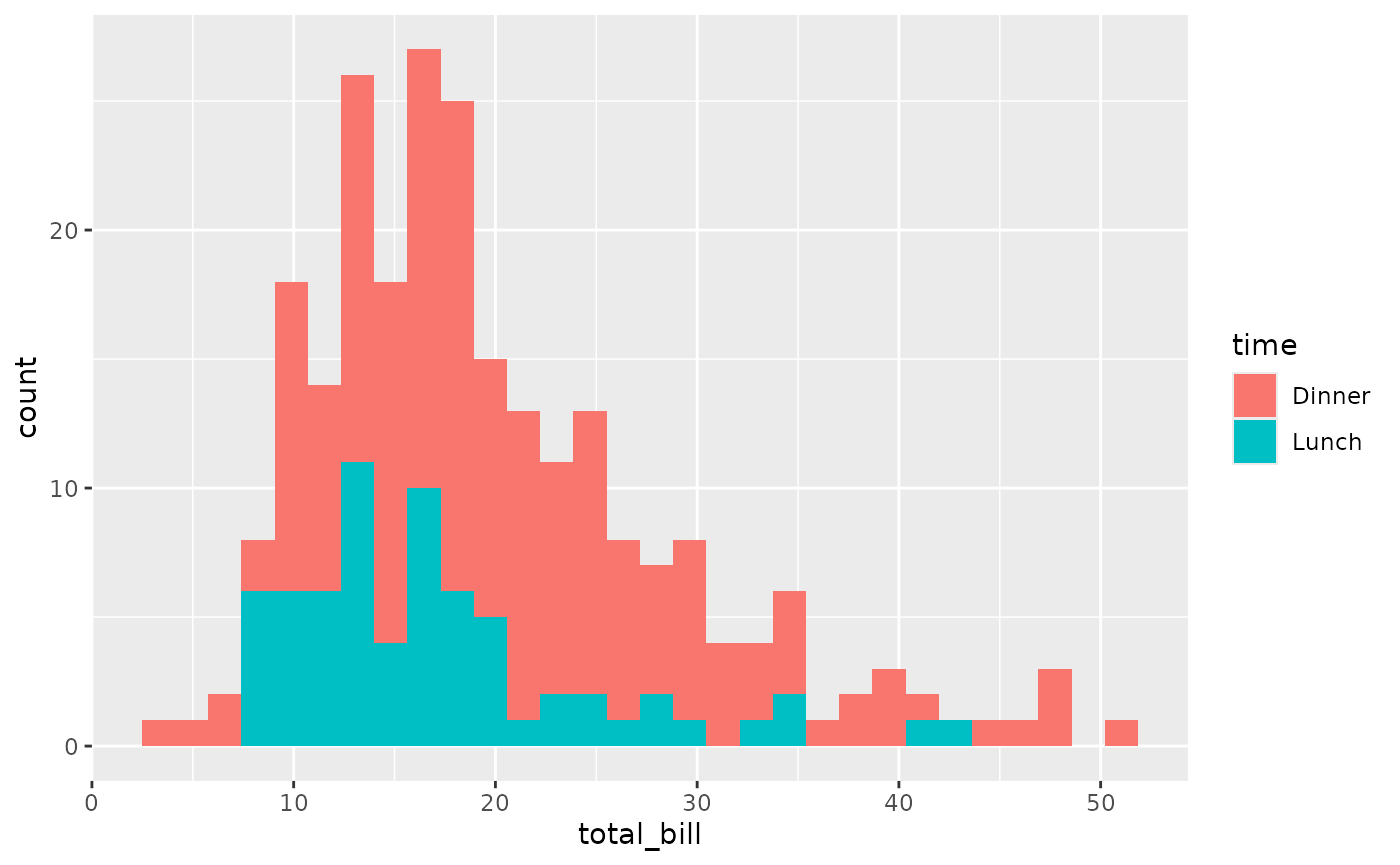#### ggally_densityDiag()

ggally_densityDiag(tips, aes(x = total_bill))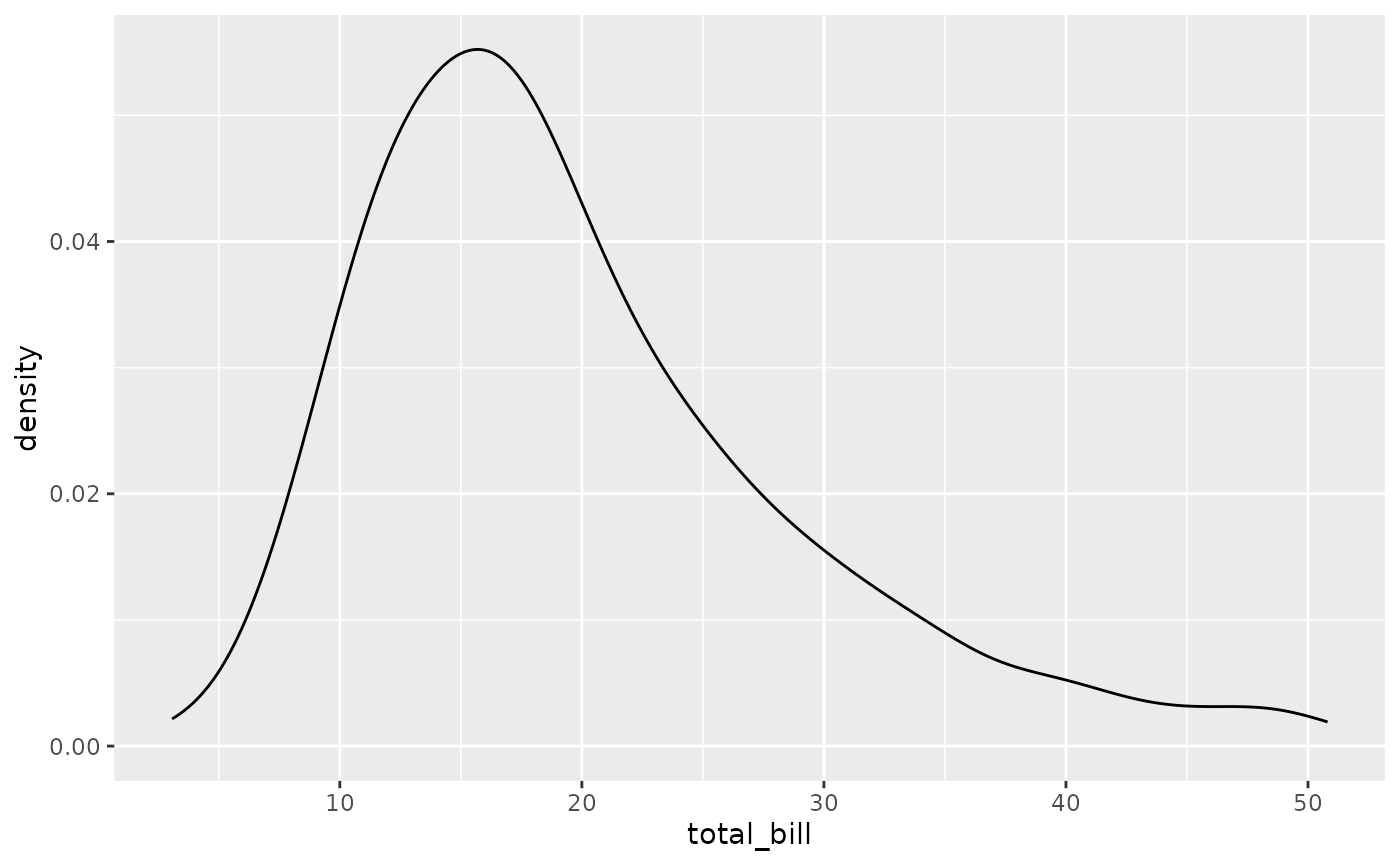ggally_densityDiag(tips, aes(x = total_bill, colour = time))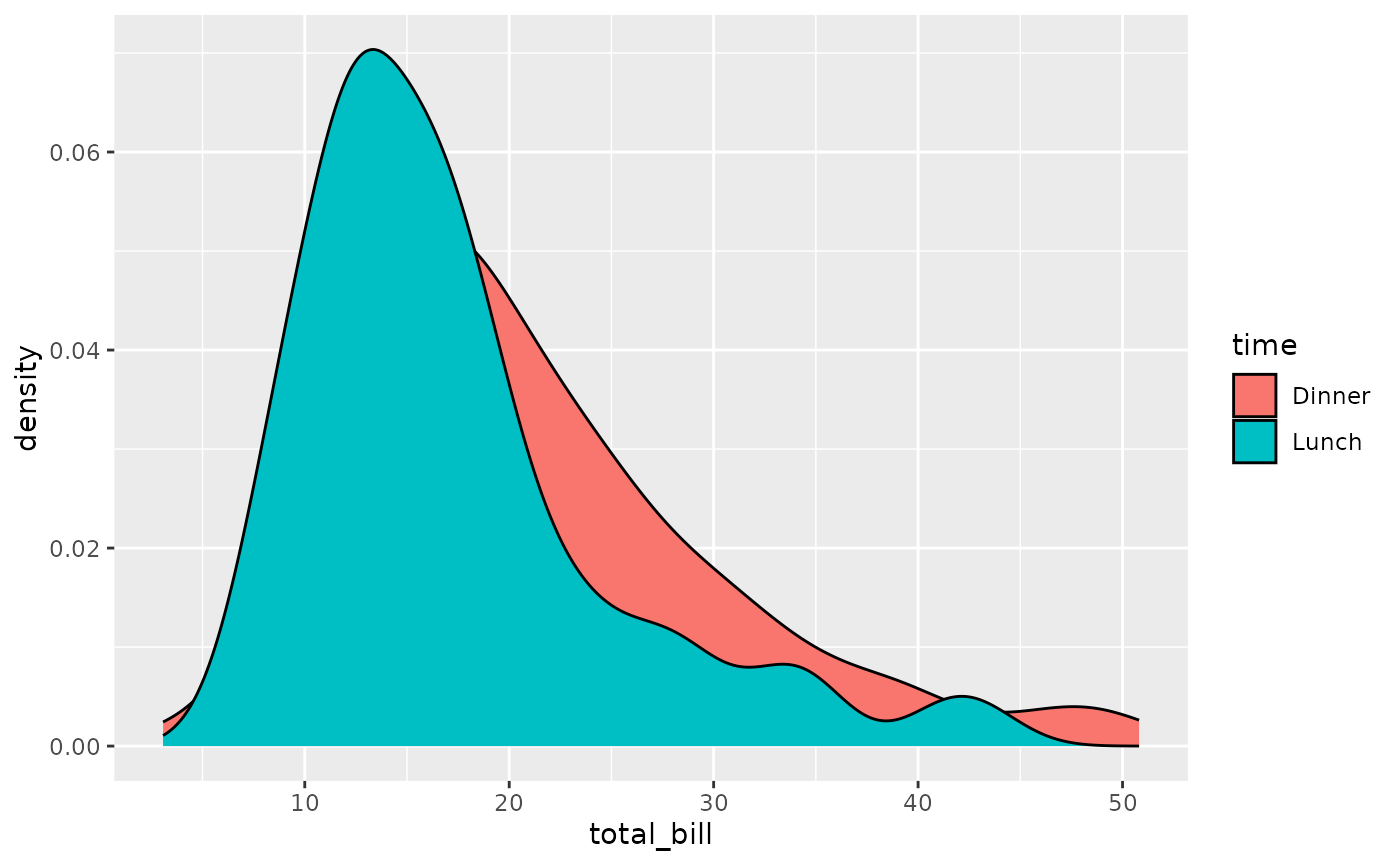### with 1x discrete variable

#### ggally_autopointDiag()

ggally_autopointDiag(tips, aes(x = day))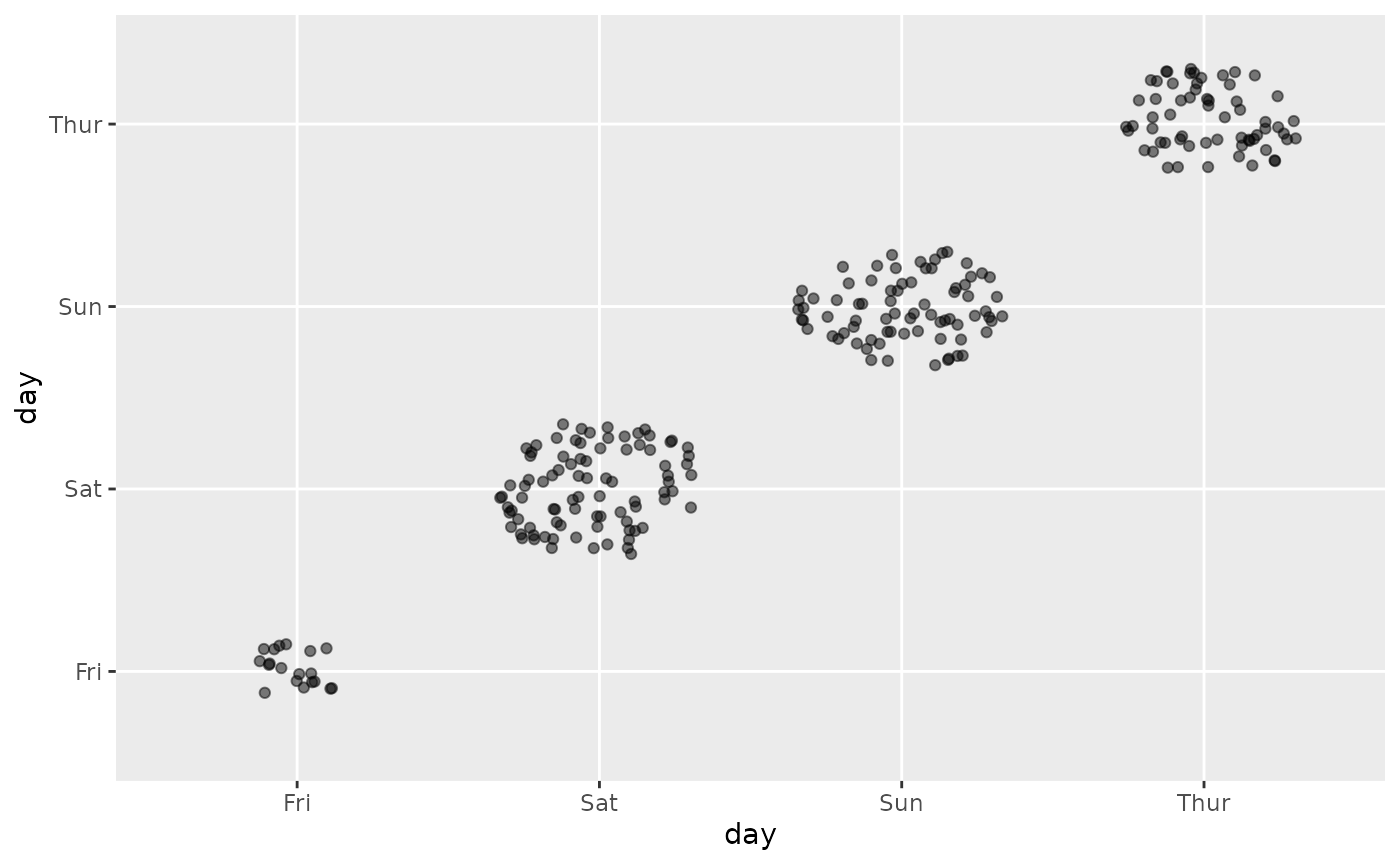ggally_autopointDiag(tips, aes(x = day, colour = time))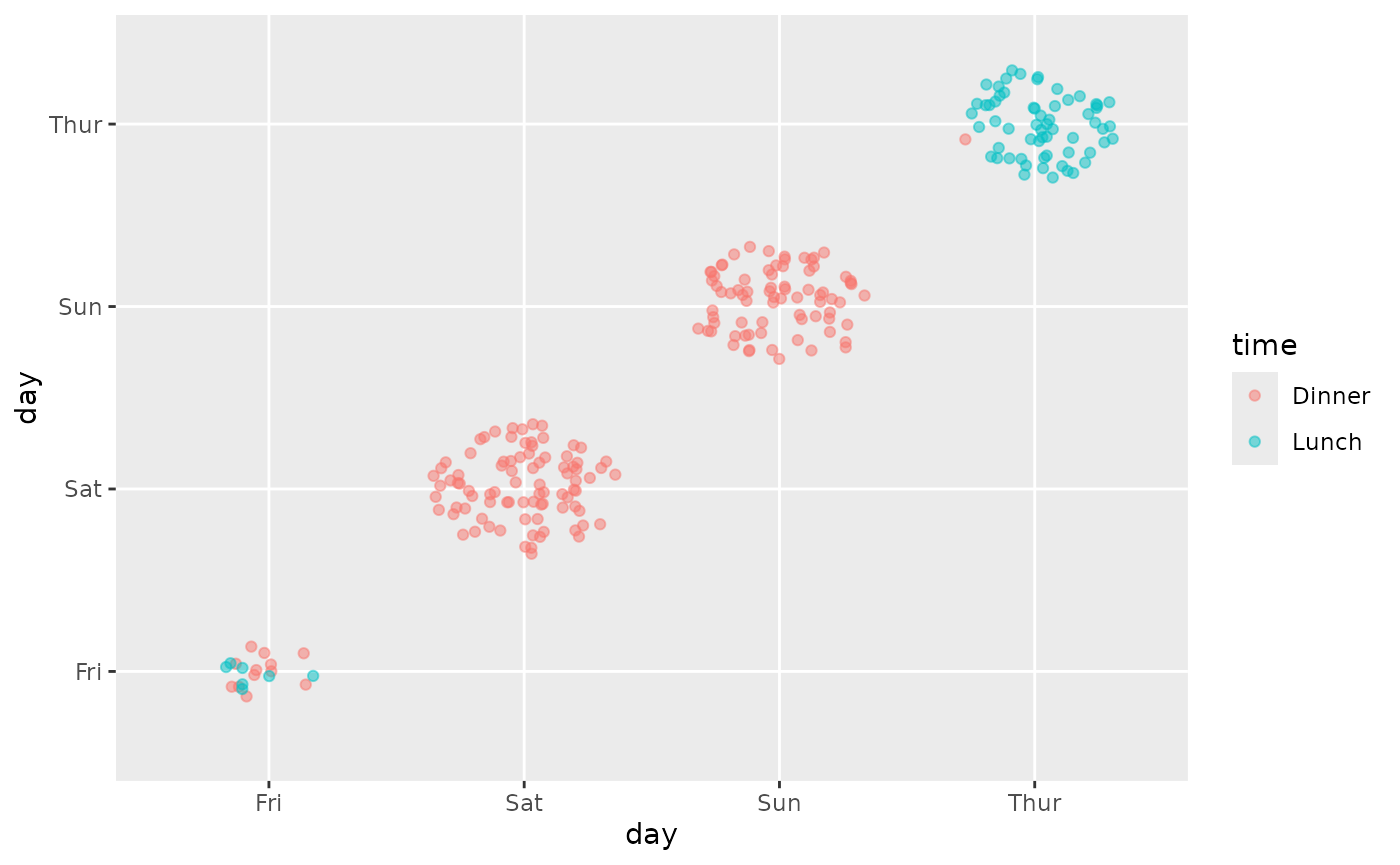#### ggally_barDiag()

ggally_barDiag(tips, aes(x = day))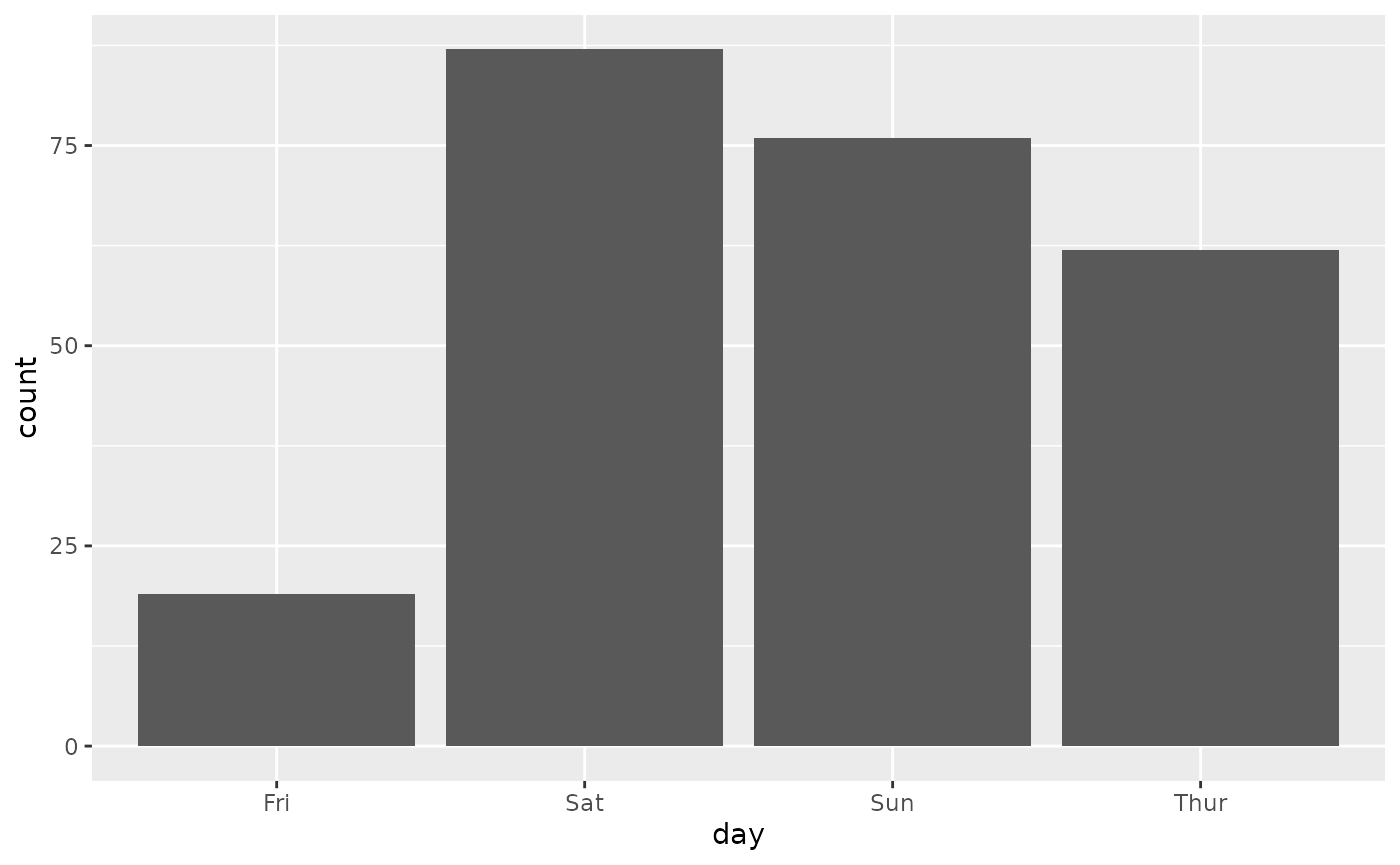ggally_barDiag(tips, aes(x = day, colour = time))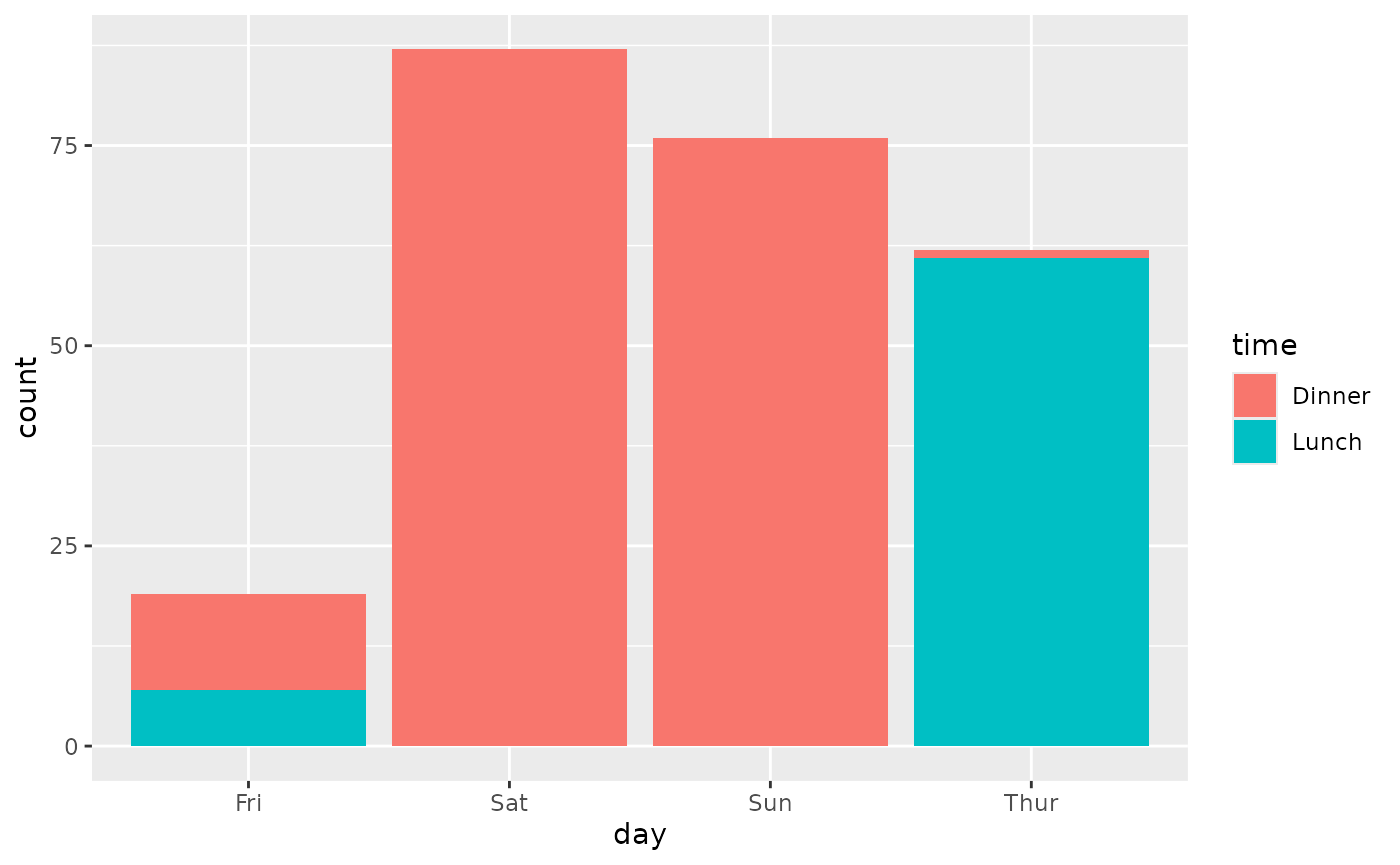#### ggally_countDiag()

ggally_countDiag(tips, aes(x = day))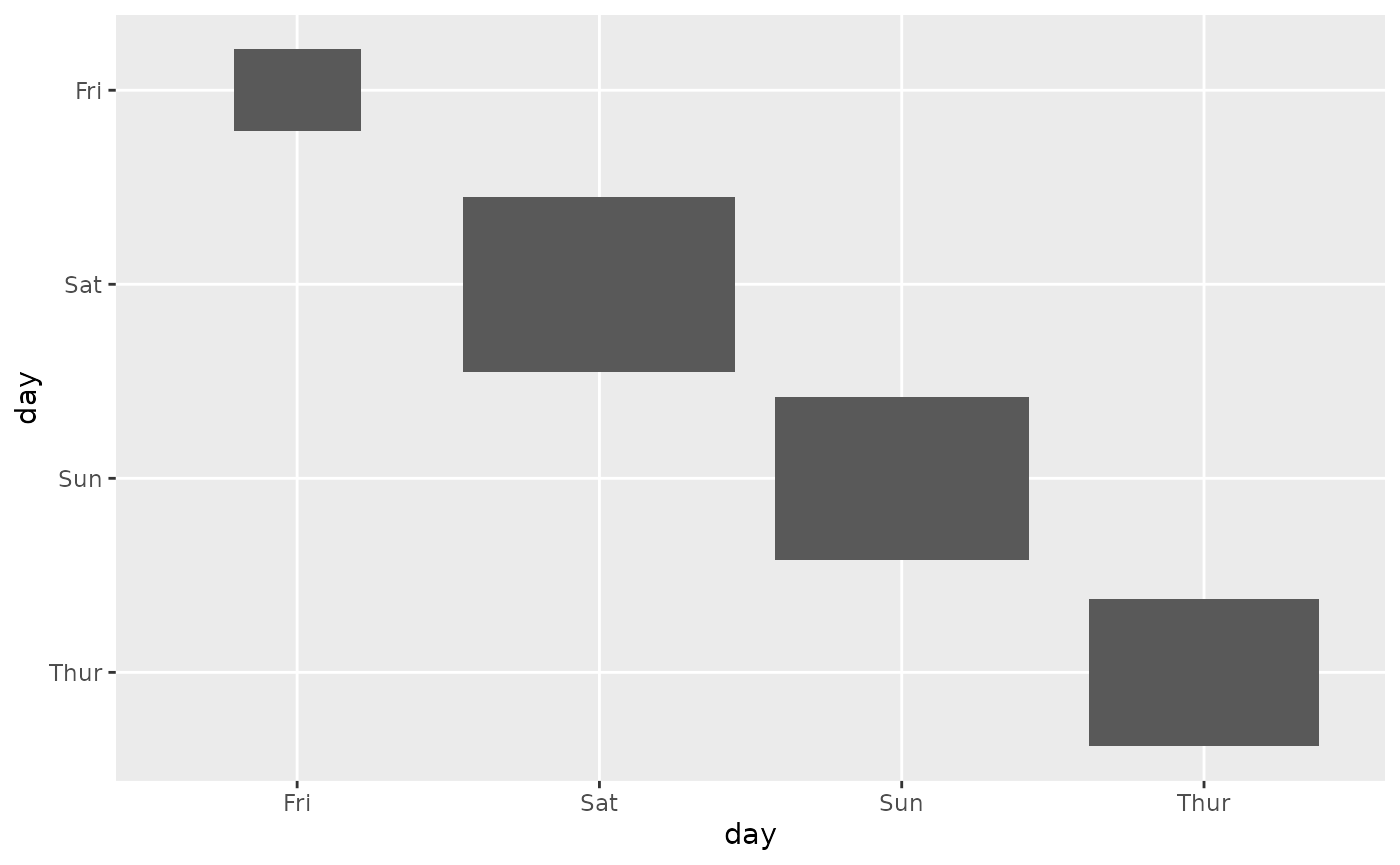ggally_countDiag(tips, aes(x = day, colour = time))#### ggally_densityDiag()

ggally_densityDiag(tips, aes(x = day))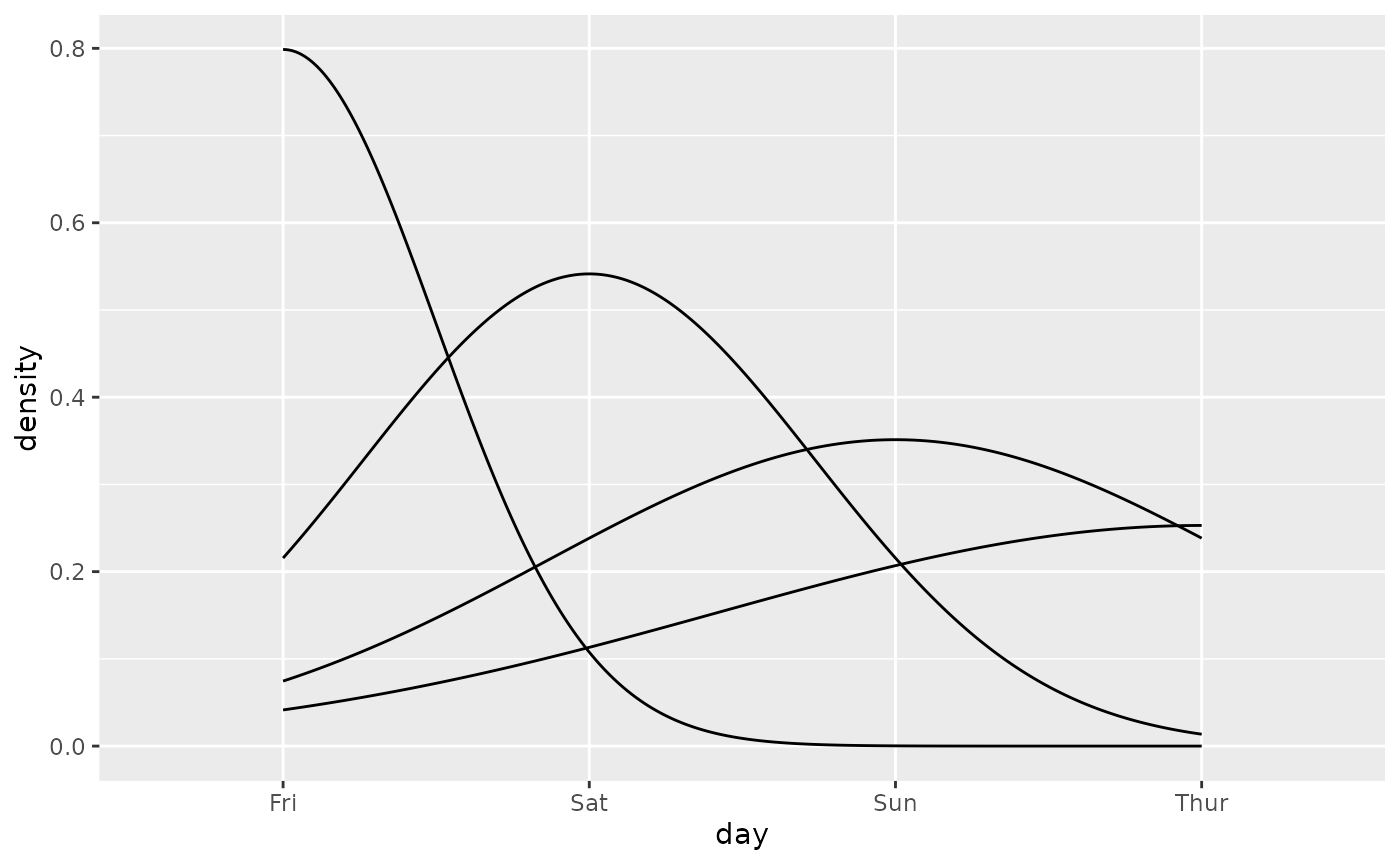ggally_densityDiag(tips, aes(x = day, colour = time))
#> Warning: Groups with fewer than two data points have been dropped.
#> Warning in max(ids, na.rm = TRUE): no non-missing arguments to max; returning
#> -Inf• ggally_statistic() and ggally_text() to display custom text
• ggally_blank() and ggally_blankDiag() for blank plot
• ggally_na() and ggally_naDiag() to display a large NA